# 25.1.9. Raster analysis¶

## 25.1.9.1. Cell stack percent rank from value¶

Calculates the cell-wise percentrank value of a stack of rasters based on a single input value and writes them to an output raster.

At each cell location, the specified value is ranked among the respective values in the stack of all overlaid and sorted cell values from the input rasters. For values outside of the stack value distribution, the algorithm returns NoData because the value cannot be ranked among the cell values.

There are two methods for percentile calculation:

• Inclusive linear interpolation (PERCENTRANK.INC)

• Exclusive linear interpolation (PERCENTRANK.EXC)

The linear interpolation method return the unique percent rank for different values. Both interpolation methods follow their counterpart methods implemented by LibreOffice or Microsoft Excel.

The output raster’s extent and resolution is defined by a reference raster. Input raster layers that do not match the cell size of the reference raster layer will be resampled using nearest neighbor resampling. NoData values in any of the input layers will result in a NoData cell output if the “Ignore NoData values” parameter is not set. The output raster data type will always be `Float32`.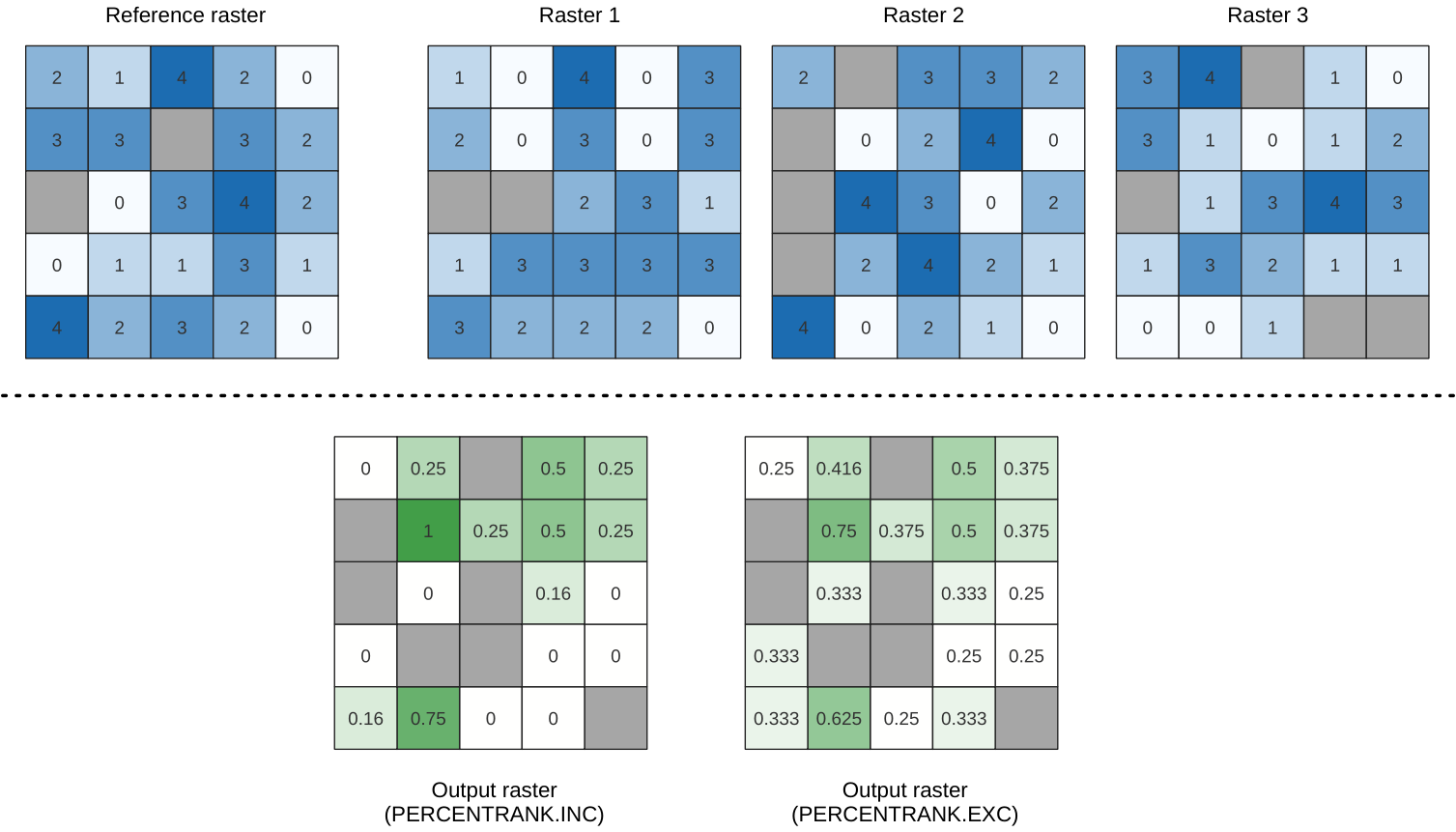Fig. 25.10 Percent ranking Value = 1. `NoData` cells (grey) are ignored.

### 25.1.9.1.1. Parameters¶

#### 25.1.9.1.1.1. Basic parameters¶

Label

Name

Type

Description

Input layers

`INPUT`

[raster] [list]

Raster layers to evaluate. If multiband rasters are used in the data raster stack, the algorithm will always perform the analysis on the first band of the rasters

Method

`METHOD`

[enumeration]

Default: 0

Method for percentile calculation:

• 0 — Inclusive linear interpolation (PERCENTRANK.INC)

• 1 — Exclusive linear interpolation (PERCENTRANK.EXC)

Value

`VALUE`

[number]

Default: 10.0

Value to rank among the respective values in the stack of all overlaid and sorted cell values from the input rasters

Ignore NoData values

`IGNORE_NODATA`

[boolean]

Default: True

If unchecked, any NoData cells in the input layers will result in a NoData cell in the output raster

Reference layer

`REFERENCE_LAYER`

[raster]

The reference layer for the output layer creation (extent, CRS, pixel dimensions)

Output layer

`OUTPUT`

[same as input]

Default: `[Save to temporary file]`

Specification of the output raster. One of:

• Save to a Temporary File

• Save to File…

Label

Name

Type

Description

Output no data value

`OUTPUT_NODATA_VALUE`

[number]

Default: -9999.0

Value to use for nodata in the output layer

### 25.1.9.1.2. Outputs¶

Label

Name

Type

Description

Output layer

`OUTPUT`

[raster]

Output raster layer containing the result

CRS authority identifier

`CRS_AUTHID`

[string]

The coordinate reference system of the output raster layer

Extent

`EXTENT`

[string]

The spatial extent of the output raster layer

Width in pixels

`WIDTH_IN_PIXELS`

[integer]

The number of columns in the output raster layer

Height in pixels

`HEIGHT_IN_PIXELS`

[integer]

The number of rows in the output raster layer

Total pixel count

`TOTAL_PIXEL_COUNT`

[integer]

The count of pixels in the output raster layer

### 25.1.9.1.3. Python code¶

Algorithm ID: `native:cellstackpercentrankfromvalue`

```import processing
processing.run("algorithm_id", {parameter_dictionary})
```

The algorithm id is displayed when you hover over the algorithm in the Processing Toolbox. The parameter dictionary provides the parameter NAMEs and values. See Using processing algorithms from the console for details on how to run processing algorithms from the Python console.

## 25.1.9.2. Cell stack percentile¶

Calculates the cell-wise percentile value of a stack of rasters and writes the results to an output raster. The percentile to return is determined by the percentile input value (ranges between 0 and 1). At each cell location, the specified percentile is obtained using the respective value from the stack of all overlaid and sorted cell values of the input rasters.

There are three methods for percentile calculation:

• Nearest rank: returns the value that is nearest to the specified percentile

• Inclusive linear interpolation (PERCENTRANK.INC)

• Exclusive linear interpolation (PERCENTRANK.EXC)

The linear interpolation methods return the unique values for different percentiles. Both interpolation methods follow their counterpart methods implemented by LibreOffice or Microsoft Excel.

The output raster’s extent and resolution is defined by a reference raster. Input raster layers that do not match the cell size of the reference raster layer will be resampled using nearest neighbor resampling. NoData values in any of the input layers will result in a NoData cell output if the “Ignore NoData values” parameter is not set. The output raster data type will always be `Float32`.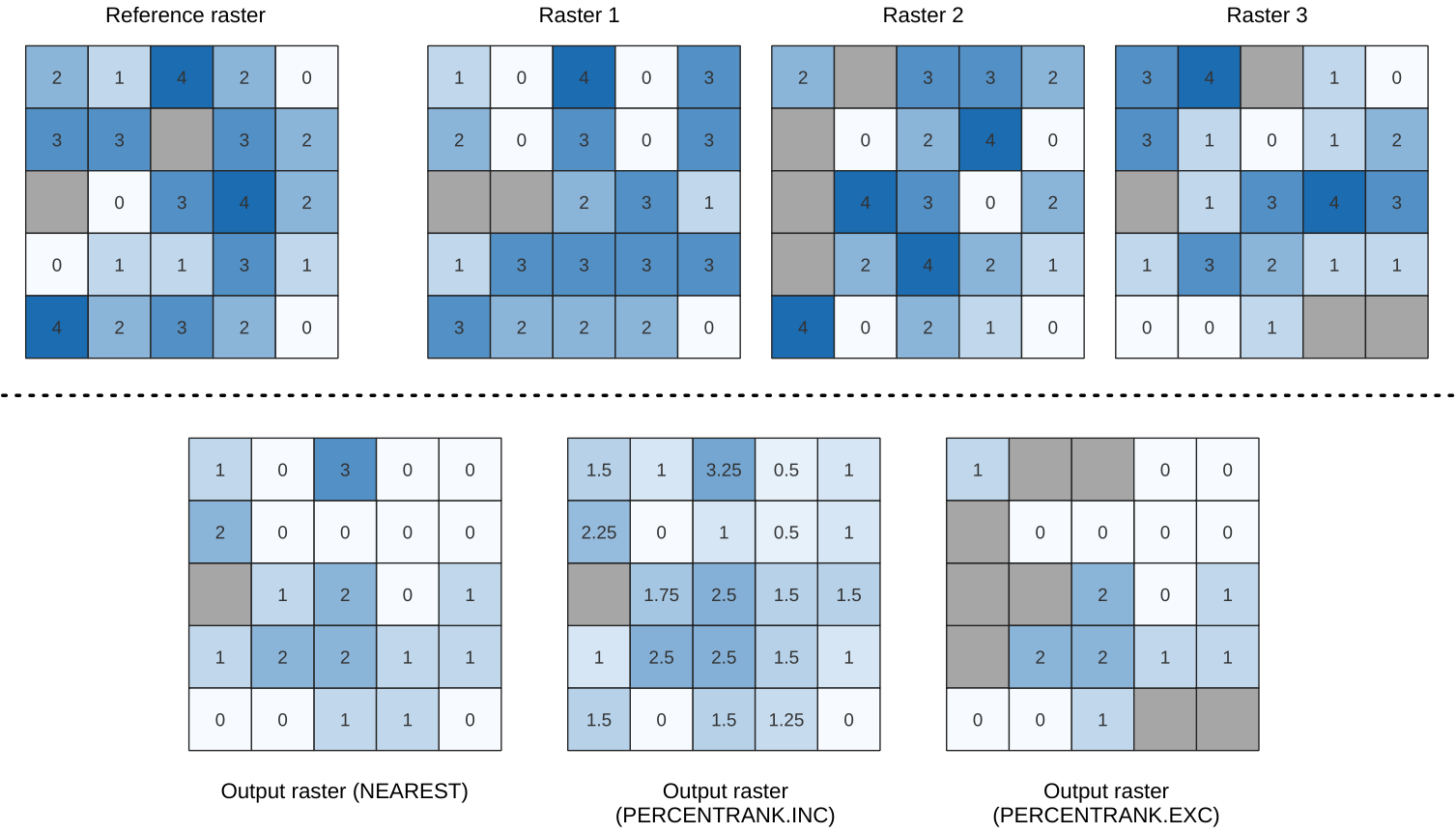Fig. 25.11 Percentile = 0.25. `NoData` cells (grey) are ignored.

### 25.1.9.2.1. Parameters¶

#### 25.1.9.2.1.1. Basic parameters¶

Label

Name

Type

Description

Input layers

`INPUT`

[raster] [list]

Raster layers to evaluate. If multiband rasters are used in the data raster stack, the algorithm will always perform the analysis on the first band of the rasters

Method

`METHOD`

[enumeration]

Default: 0

Method for percentile calculation:

• 0 — Nearest rank: returns the value that is nearest to the specified percentile

• 1 — Inclusive linear interpolation (PERCENTILE.INC)

• 2 — Exclusive linear interpolation (PERCENTILE.EXC)

Percentile

`VALUE`

[number]

Default: 0.25

Value to rank among the respective values in the stack of all overlaid and sorted cell values from the input rasters. Between 0 and 1.

Ignore NoData values

`IGNORE_NODATA`

[boolean]

Default: True

If unchecked, any NoData cells in the input layers will result in a NoData cell in the output raster

Reference layer

`REFERENCE_LAYER`

[raster]

The reference layer for the output layer creation (extent, CRS, pixel dimensions)

Output layer

`OUTPUT`

[same as input]

Default: `[Save to temporary file]`

Specification of the output raster. One of:

• Save to a Temporary File

• Save to File…

Label

Name

Type

Description

Output no data value

`OUTPUT_NODATA_VALUE`

[number]

Default: -9999.0

Value to use for nodata in the output layer

### 25.1.9.2.2. Outputs¶

Label

Name

Type

Description

Output layer

`OUTPUT`

[raster]

Output raster layer containing the result

CRS authority identifier

`CRS_AUTHID`

[string]

The coordinate reference system of the output raster layer

Extent

`EXTENT`

[string]

The spatial extent of the output raster layer

Width in pixels

`WIDTH_IN_PIXELS`

[integer]

The number of columns in the output raster layer

Height in pixels

`HEIGHT_IN_PIXELS`

[integer]

The number of rows in the output raster layer

Total pixel count

`TOTAL_PIXEL_COUNT`

[integer]

The count of pixels in the output raster layer

### 25.1.9.2.3. Python code¶

Algorithm ID: `native:cellstackpercentile`

```import processing
processing.run("algorithm_id", {parameter_dictionary})
```

The algorithm id is displayed when you hover over the algorithm in the Processing Toolbox. The parameter dictionary provides the parameter NAMEs and values. See Using processing algorithms from the console for details on how to run processing algorithms from the Python console.

## 25.1.9.3. cell stack percentrank from raster layer¶

Calculates the cell-wise percentrank value of a stack of rasters based on an input value raster and writes them to an output raster.

At each cell location, the current value of the value raster is ranked among the respective values in the stack of all overlaid and sorted cell values of the input rasters. For values outside of the the stack value distribution, the algorithm returns NoData because the value cannot be ranked among the cell values.

There are two methods for percentile calculation:

• Inclusive linear interpolation (PERCENTRANK.INC)

• Exclusive linear interpolation (PERCENTRANK.EXC)

The linear interpolation methods return the unique values for different percentiles. Both interpolation methods follow their counterpart methods implemented by LibreOffice or Microsoft Excel.

The output raster’s extent and resolution is defined by a reference raster. Input raster layers that do not match the cell size of the reference raster layer will be resampled using nearest neighbor resampling. NoData values in any of the input layers will result in a NoData cell output if the “Ignore NoData values” parameter is not set. The output raster data type will always be `Float32`.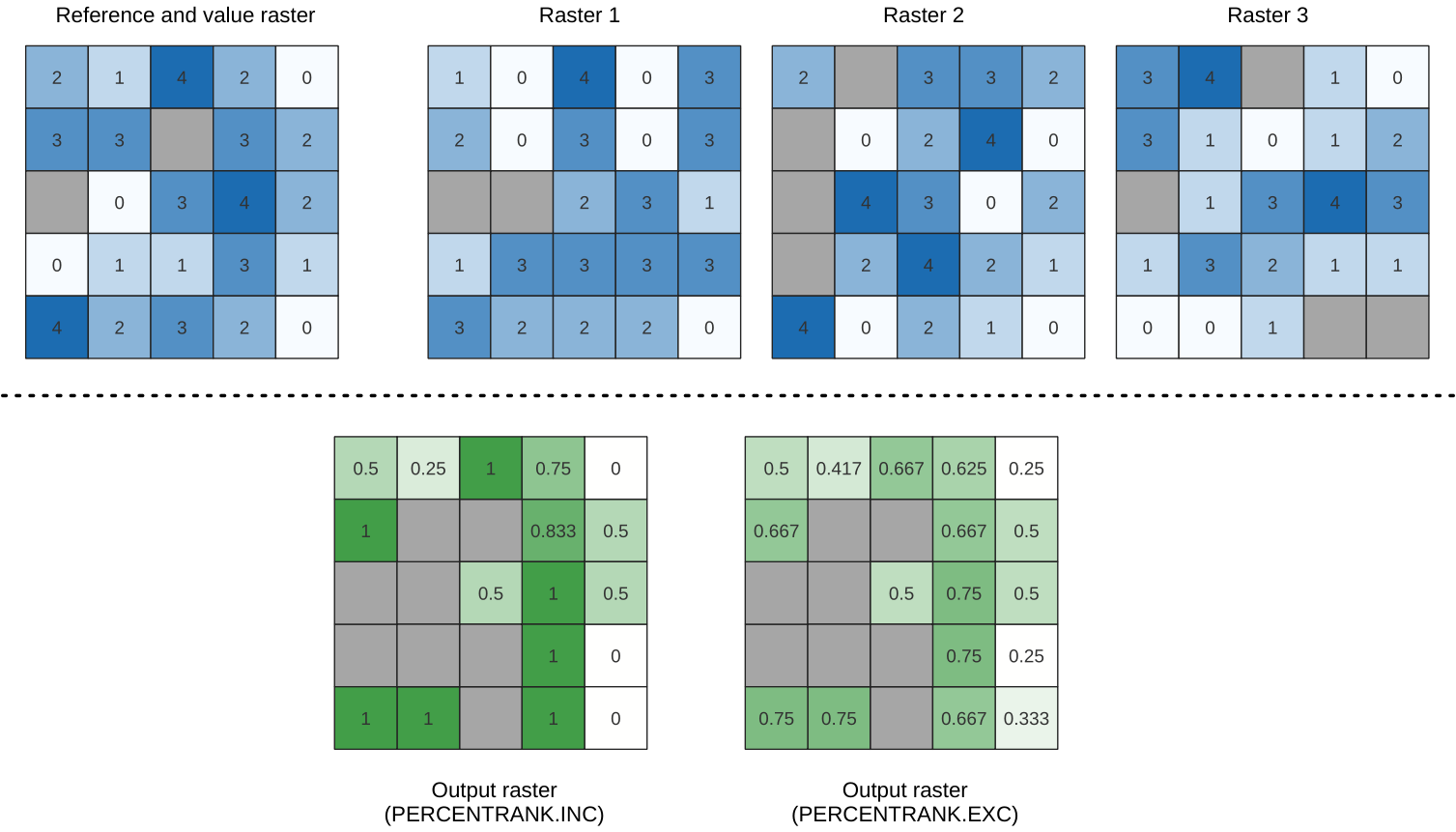Fig. 25.12 Ranking the value raster layer cells. `NoData` cells (grey) are ignored.

### 25.1.9.3.1. Parameters¶

#### 25.1.9.3.1.1. Basic parameters¶

Label

Name

Type

Description

Input layers

`INPUT`

[raster] [list]

Raster layers to evaluate. If multiband rasters are used in the data raster stack, the algorithm will always perform the analysis on the first band of the rasters

Value raster layer

`INPUT_VALUE_RASTER`

[raster]

The layer to rank the values among the stack of all overlaid layers

Value raster band

`VALUE_RASTER_BAND`

[integer]

Default: 1

Band of the “value raster layer” to compare to

Method

`METHOD`

[enumeration]

Default: 0

Method for percentile calculation:

• 0 — Inclusive linear interpolation (PERCENTRANK.INC)

• 1 — Exclusive linear interpolation (PERCENTRANK.EXC)

Ignore NoData values

`IGNORE_NODATA`

[boolean]

Default: True

If unchecked, any NoData cells in the input layers will result in a NoData cell in the output raster

Reference layer

`REFERENCE_LAYER`

[raster]

The reference layer for the output layer creation (extent, CRS, pixel dimensions)

Output layer

`OUTPUT`

[same as input]

Default: `[Save to temporary file]`

Specification of the output raster. One of:

• Save to a Temporary File

• Save to File…

Label

Name

Type

Description

Output no data value

`OUTPUT_NODATA_VALUE`

[number]

Default: -9999.0

Value to use for nodata in the output layer

### 25.1.9.3.2. Outputs¶

Label

Name

Type

Description

Output layer

`OUTPUT`

[raster]

Output raster layer containing the result

CRS authority identifier

`CRS_AUTHID`

[string]

The coordinate reference system of the output raster layer

Extent

`EXTENT`

[string]

The spatial extent of the output raster layer

Width in pixels

`WIDTH_IN_PIXELS`

[integer]

The number of columns in the output raster layer

Height in pixels

`HEIGHT_IN_PIXELS`

[integer]

The number of rows in the output raster layer

Total pixel count

`TOTAL_PIXEL_COUNT`

[integer]

The count of pixels in the output raster layer

### 25.1.9.3.3. Python code¶

Algorithm ID: `native:cellstackpercentrankfromrasterlayer`

```import processing
processing.run("algorithm_id", {parameter_dictionary})
```

The algorithm id is displayed when you hover over the algorithm in the Processing Toolbox. The parameter dictionary provides the parameter NAMEs and values. See Using processing algorithms from the console for details on how to run processing algorithms from the Python console.

## 25.1.9.4. Cell statistics¶

Computes per-cell statistics based on input raster layers and for each cell writes the resulting statistics to an output raster. At each cell location, the output value is defined as a function of all overlaid cell values of the input rasters.

By default, a NoData cell in ANY of the input layers will result in a NoData cell in the output raster. If the Ignore NoData values option is checked, then NoData inputs will be ignored in the statistic calculation. This may result in NoData output for locations where all cells are NoData.

The Reference layer parameter specifies an existing raster layer to use as a reference when creating the output raster. The output raster will have the same extent, CRS, and pixel dimensions as this layer.

Calculation details: Input raster layers that do not match the cell size of the reference raster layer will be resampled using `nearest neighbor resampling`. The output raster data type will be set to the most complex data type present in the input datasets except when using the functions `Mean`, `Standard deviation` and `Variance` (data type is always `Float32` or `Float64` depending on input float type) or `Count` and `Variety` (data type is always `Int32`).

• `Count`: The count statistic will always result in the number of cells without NoData values at the current cell location.

• `Median`: If the number of input layers is even, the median will be calculated as the arithmetic mean of the two middle values of the ordered cell input values.

• `Minority/Majority`: If no unique minority or majority could be found, the result is NoData, except all input cell values are equal.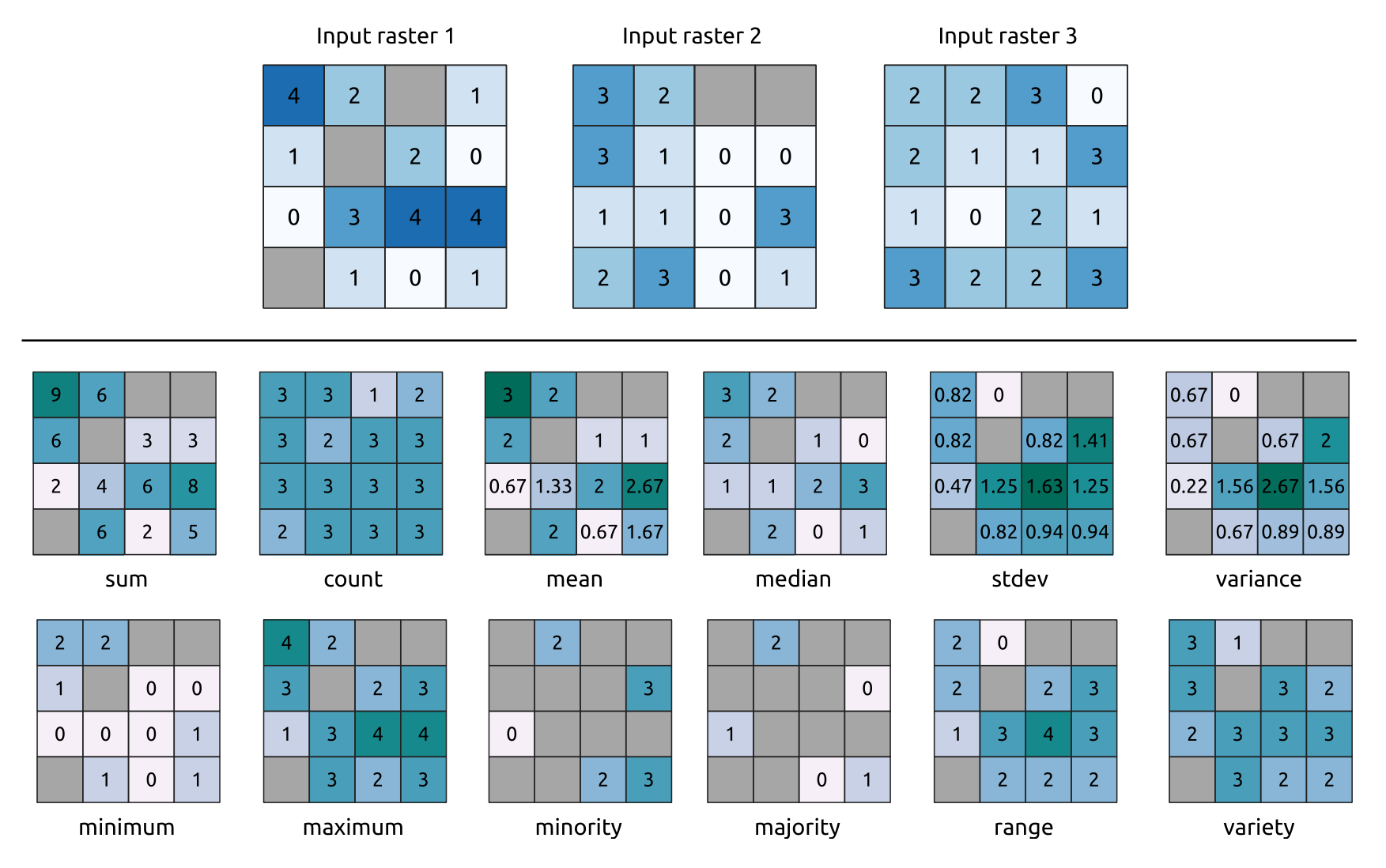Fig. 25.13 Example with all the statistic functions. `NoData` cells (grey) are taken into account.

### 25.1.9.4.1. Parameters¶

#### 25.1.9.4.1.1. Basic parameters¶

Label

Name

Type

Description

Input layers

`INPUT`

[raster] [list]

Input raster layers

Statistic

`STATISTIC`

[enumeration]

Default: 0

Available statistics. Options:

• 0 — Sum

• 1 — Count

• 2 — Mean

• 3 — Median

• 4 — Standard deviation

• 5 — Variance

• 6 — Minimum

• 7 — Maximum

• 8 — Minority (least common value)

• 9 — Majority (most common value)

• 10 — Range (max - min)

• 11 — Variety (unique value count)

Ignore NoData values

`IGNORE_NODATA`

[boolean]

Default: True

Calculate statistics also for all cells stacks, ignoring NoData occurrence.

Reference layer

`REF_LAYER`

[raster]

The reference layer to create the output layer from (extent, CRS, pixel dimensions)

Output layer

`OUTPUT`

[same as input]

Default: `[Save to temporary file]`

Specification of the output raster. One of:

• Save to a Temporary File

• Save to File…

Label

Name

Type

Description

Output no data value

Optional

`OUTPUT_NO_DATA_VALUE`

[number]

Default: -9999.0

Value to use for nodata in the output layer

### 25.1.9.4.2. Outputs¶

Label

Name

Type

Description

CRS authority identifier

`CRS_AUTHID`

[crs]

The coordinate reference system of the output raster layer

Extent

`EXTENT`

[extent]

The spatial extent of the output raster layer

Height in pixels

`HEIGHT_IN_PIXELS`

[integer]

The number of rows in the output raster layer

Output raster

`OUTPUT`

[raster]

Output raster layer containing the result

Total pixel count

`TOTAL_PIXEL_COUNT`

[integer]

The count of pixels in the output raster layer

Width in pixels

`WIDTH_IN_PIXELS`

[integer]

The number of columns in the output raster layer

### 25.1.9.4.3. Python code¶

Algorithm ID: `native:cellstatistics`

```import processing
processing.run("algorithm_id", {parameter_dictionary})
```

The algorithm id is displayed when you hover over the algorithm in the Processing Toolbox. The parameter dictionary provides the parameter NAMEs and values. See Using processing algorithms from the console for details on how to run processing algorithms from the Python console.

## 25.1.9.5. Equal to frequency¶

Evaluates on a cell-by-cell basis the frequency (number of times) the values of an input stack of rasters are equal to the value of a value layer. The output raster extent and resolution are defined by the input raster layer and is always of `Int32` type.

If multiband rasters are used in the data raster stack, the algorithm will always perform the analysis on the first band of the rasters - use GDAL to use other bands in the analysis. The output NoData value can be set manually.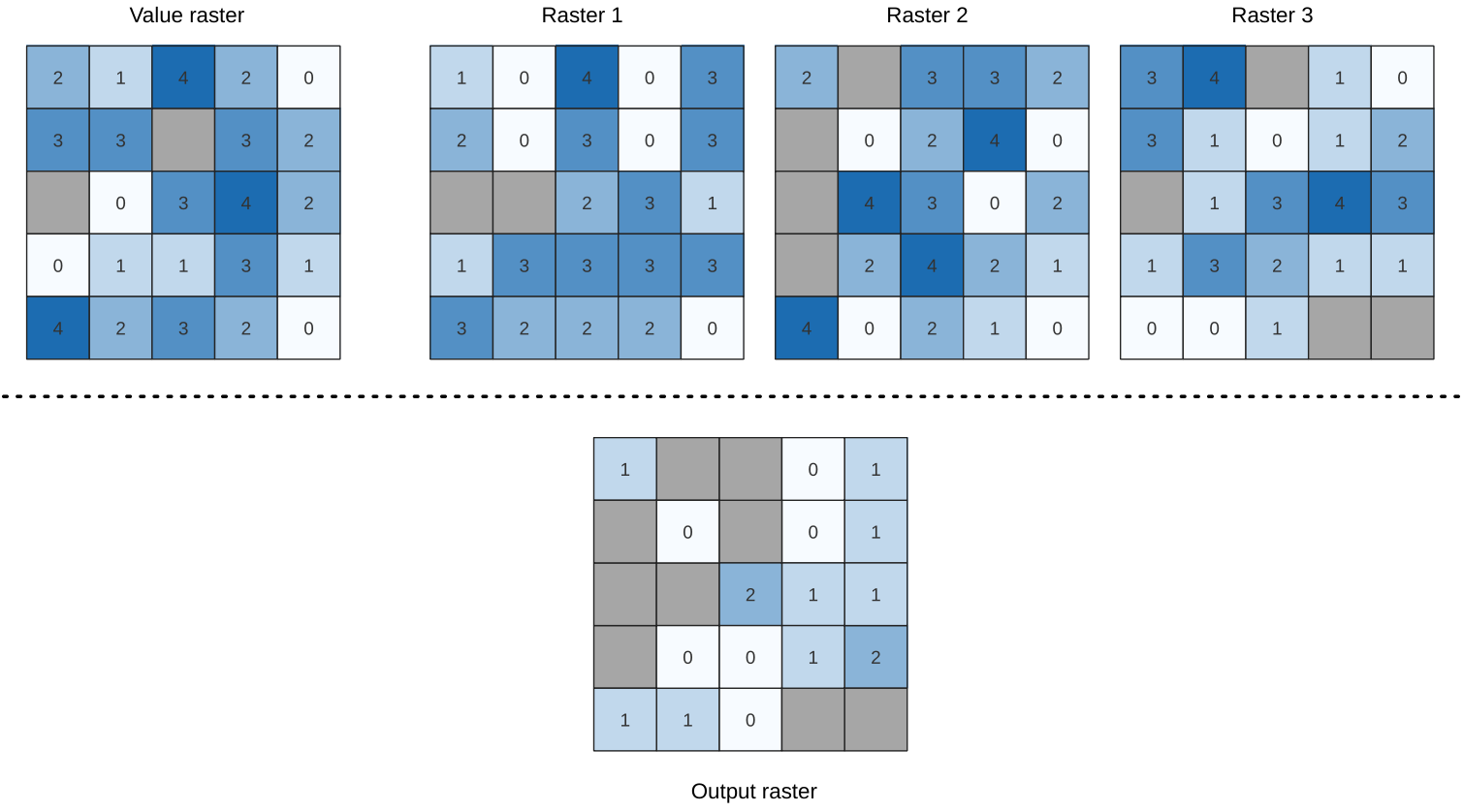Fig. 25.14 For each cell in the output raster, the value represents the number of times that the corresponding cells in the list of rasters are the same as the value raster. `NoData` cells (grey) are taken into account.

### 25.1.9.5.1. Parameters¶

#### 25.1.9.5.1.1. Basic parameters¶

Label

Name

Type

Description

Input value raster

`INPUT_VALUE_RASTER`

[raster]

The input value layer serves as reference layer for the sample layers

Value raster band

`INPUT_VALUE_RASTER_BAND`

[raster band]

Default: The first band of the raster layer

Select the band you want to use as sample

Input raster layers

`INPUT_RASTERS`

[raster] [list]

Raster layers to evaluate. If multiband rasters are used in the data raster stack, the algorithm will always perform the analysis on the first band of the rasters

Ignore NoData values

`IGNORE_NODATA`

[boolean]

Default: False

If unchecked, any NoData cells in the value raster or the data layer stack will result in a NoData cell in the output raster

Output layer

`OUTPUT`

[same as input]

Default: `[Save to temporary file]`

Specification of the output raster. One of:

• Save to a Temporary File

• Save to File…

Label

Name

Type

Description

Output no data value

Optional

`OUTPUT_NO_DATA_VALUE`

[number]

Default: -9999.0

Value to use for nodata in the output layer

### 25.1.9.5.2. Outputs¶

Label

Name

Type

Description

Output layer

`OUTPUT`

[raster]

Output raster layer containing the result

CRS authority identifier

`CRS_AUTHID`

[string]

The coordinate reference system of the output raster layer

Extent

`EXTENT`

[string]

The spatial extent of the output raster layer

Count of cells with equal value occurrences

`FOUND_LOCATIONS_COUNT`

[number]

Height in pixels

`HEIGHT_IN_PIXELS`

[number]

The number of rows in the output raster layer

Total pixel count

`TOTAL_PIXEL_COUNT`

[integer]

The count of pixels in the output raster layer

Mean frequency at valid cell locations

`MEAN_FREQUENCY_PER_LOCATION`

[number]

Count of value occurrences

`OCCURRENCE_COUNT`

[number]

Width in pixels

`WIDTH_IN_PIXELS`

[integer]

The number of columns in the output raster layer

### 25.1.9.5.3. Python code¶

Algorithm ID: `native:equaltofrequency`

```import processing
processing.run("algorithm_id", {parameter_dictionary})
```

The algorithm id is displayed when you hover over the algorithm in the Processing Toolbox. The parameter dictionary provides the parameter NAMEs and values. See Using processing algorithms from the console for details on how to run processing algorithms from the Python console.

## 25.1.9.6. Fuzzify raster (gaussian membership)¶

Transforms an input raster to a fuzzified raster by assigning a membership value to each pixel, using a Gaussian membership function. Membership values range from 0 to 1. In the fuzzified raster, a value of 0 implies no membership of the defined fuzzy set, whereas a value of 1 means full membership. The gaussian membership function is defined as, where f1 is the spread and f2 the midpoint.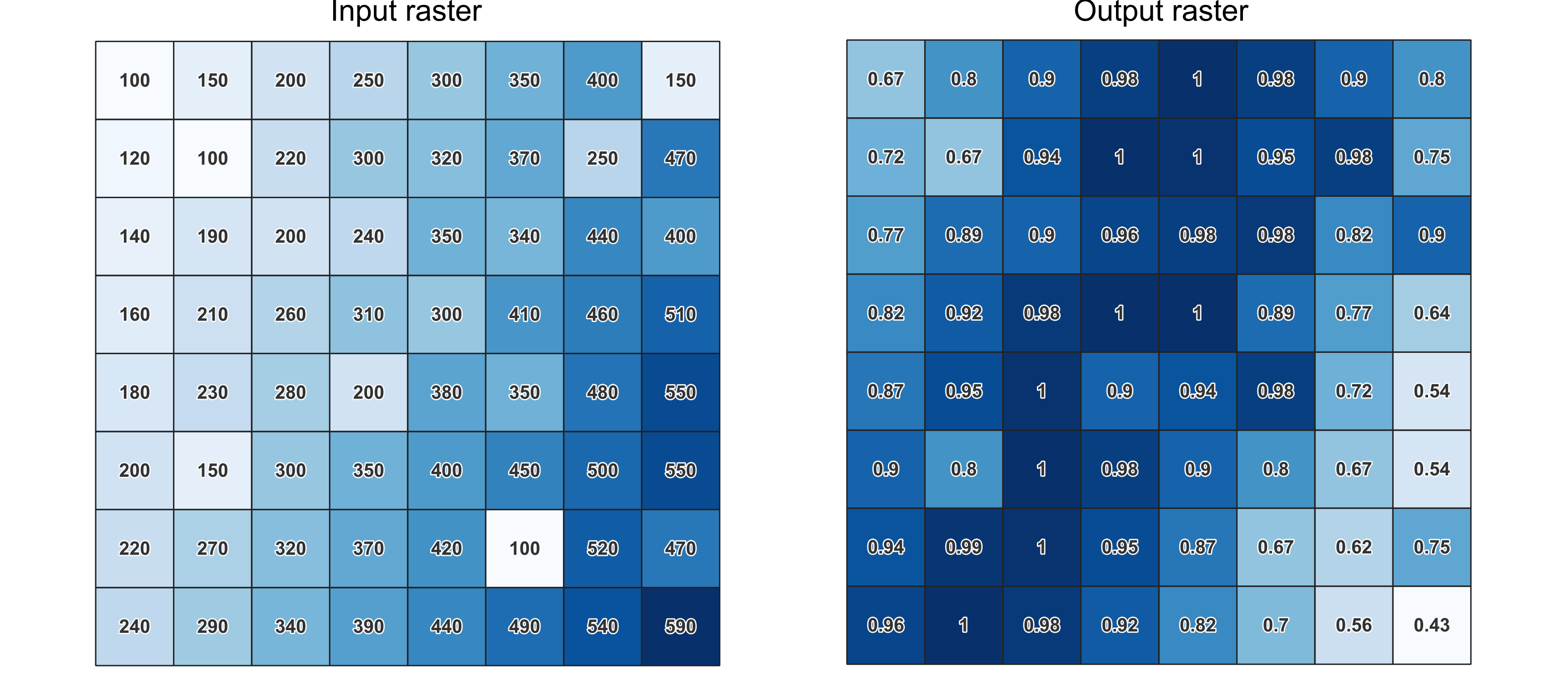Fig. 25.15 Fuzzify raster example. Input raster source: Land Tirol - data.tirol.gv.at.

### 25.1.9.6.1. Parameters¶

Label

Name

Type

Description

Input Raster

`INPUT`

[raster]

Input raster layer

Band Number

`BAND`

[raster band]

Default: The first band of the raster layer

If the raster is multiband, choose the band that you want to fuzzify.

Function midpoint

`FUZZYMIDPOINT`

[number]

Default: 10

Midpoint of the gaussian function

`FUZZYSPREAD`

[number]

Default: 0.01

Fuzzified raster

`OUTPUT`

[same as input]

Default: `[Save to temporary file]`

Specification of the output raster. One of:

• Save to a Temporary File

• Save to File…

### 25.1.9.6.2. Outputs¶

Label

Name

Type

Description

Fuzzified raster

`OUTPUT`

[same as input]

Output raster layer containing the result

CRS authority identifier

`CRS_AUTHID`

[crs]

The coordinate reference system of the output raster layer

Extent

`EXTENT`

[extent]

The spatial extent of the output raster layer

Width in pixels

`WIDTH_IN_PIXELS`

[integer]

The number of columns in the output raster layer

Height in pixels

`HEIGHT_IN_PIXELS`

[integer]

The number of rows in the output raster layer

Total pixel count

`TOTAL_PIXEL_COUNT`

[integer]

The count of pixels in the output raster layer

### 25.1.9.6.3. Python code¶

Algorithm ID: `native:fuzzifyrastergaussianmembership`

```import processing
processing.run("algorithm_id", {parameter_dictionary})
```

The algorithm id is displayed when you hover over the algorithm in the Processing Toolbox. The parameter dictionary provides the parameter NAMEs and values. See Using processing algorithms from the console for details on how to run processing algorithms from the Python console.

## 25.1.9.7. Fuzzify raster (large membership)¶

Transforms an input raster to a fuzzified raster by assigning a membership value to each pixel, using a Large membership function. Membership values range from 0 to 1. In the fuzzified raster, a value of 0 implies no membership of the defined fuzzy set, whereas a value of 1 means full membership. The large membership function is defined as, where f1 is the spread and f2 the midpoint.

### 25.1.9.7.1. Parameters¶

Label

Name

Type

Description

Input Raster

`INPUT`

[raster]

Input raster layer

Band Number

`BAND`

[raster band]

Default: The first band of the raster layer

If the raster is multiband, choose the band that you want to fuzzify.

Function midpoint

`FUZZYMIDPOINT`

[number]

Default: 50

Midpoint of the large function

`FUZZYSPREAD`

[number]

Default: 5

Fuzzified raster

`OUTPUT`

[same as input]

Default: `[Save to temporary file]`

Specification of the output raster. One of:

• Save to a Temporary File

• Save to File…

### 25.1.9.7.2. Outputs¶

Label

Name

Type

Description

Fuzzified raster

`OUTPUT`

[same as input]

Output raster layer containing the result

CRS authority identifier

`CRS_AUTHID`

[crs]

The coordinate reference system of the output raster layer

Extent

`EXTENT`

[extent]

The spatial extent of the output raster layer

Width in pixels

`WIDTH_IN_PIXELS`

[integer]

The number of columns in the output raster layer

Height in pixels

`HEIGHT_IN_PIXELS`

[integer]

The number of rows in the output raster layer

Total pixel count

`TOTAL_PIXEL_COUNT`

[integer]

The count of pixels in the output raster layer

### 25.1.9.7.3. Python code¶

Algorithm ID: `native:fuzzifyrasterlargemembership`

```import processing
processing.run("algorithm_id", {parameter_dictionary})
```

The algorithm id is displayed when you hover over the algorithm in the Processing Toolbox. The parameter dictionary provides the parameter NAMEs and values. See Using processing algorithms from the console for details on how to run processing algorithms from the Python console.

## 25.1.9.8. Fuzzify raster (linear membership)¶

Transforms an input raster to a fuzzified raster by assigning a membership value to each pixel, using a Linear membership function. Membership values range from 0 to 1. In the fuzzified raster, a value of 0 implies no membership of the defined fuzzy set, whereas a value of 1 means full membership. The linear function is defined as, where a is the low bound and b the high bound. This equation assigns membership values using a linear transformation for pixel values between the low and high bounds. Pixels values smaller than the low bound are given 0 membership whereas pixel values greater than the high bound are given 1 membership.

### 25.1.9.8.1. Parameters¶

Label

Name

Type

Description

Input Raster

`INPUT`

[raster]

Input raster layer

Band Number

`BAND`

[raster band]

Default: The first band of the raster layer

If the raster is multiband, choose the band that you want to fuzzify.

Low fuzzy membership bound

`FUZZYLOWBOUND`

[number]

Default: 0

Low bound of the linear function

High fuzzy membership bound

`FUZZYHIGHBOUND`

[number]

Default: 1

High bound of the linear function

Fuzzified raster

`OUTPUT`

[same as input]

Default: `[Save to temporary file]`

Specification of the output raster. One of:

• Save to a Temporary File

• Save to File…

### 25.1.9.8.2. Outputs¶

Label

Name

Type

Description

Fuzzified raster

`OUTPUT`

[same as input]

Output raster layer containing the result

CRS authority identifier

`CRS_AUTHID`

[crs]

The coordinate reference system of the output raster layer

Extent

`EXTENT`

[extent]

The spatial extent of the output raster layer

Width in pixels

`WIDTH_IN_PIXELS`

[integer]

The number of columns in the output raster layer

Height in pixels

`HEIGHT_IN_PIXELS`

[integer]

The number of rows in the output raster layer

Total pixel count

`TOTAL_PIXEL_COUNT`

[integer]

The count of pixels in the output raster layer

### 25.1.9.8.3. Python code¶

Algorithm ID: `native:fuzzifyrasterlinearmembership`

```import processing
processing.run("algorithm_id", {parameter_dictionary})
```

The algorithm id is displayed when you hover over the algorithm in the Processing Toolbox. The parameter dictionary provides the parameter NAMEs and values. See Using processing algorithms from the console for details on how to run processing algorithms from the Python console.

## 25.1.9.9. Fuzzify raster (near membership)¶

Transforms an input raster to a fuzzified raster by assigning a membership value to each pixel, using a Near membership function. Membership values range from 0 to 1. In the fuzzified raster, a value of 0 implies no membership of the defined fuzzy set, whereas a value of 1 means full membership. The near membership function is defined as, where f1 is the spread and f2 the midpoint.

### 25.1.9.9.1. Parameters¶

Label

Name

Type

Description

Input Raster

`INPUT`

[raster]

Input raster layer

Band Number

`BAND`

[raster band]

Default: The first band of the raster layer

If the raster is multiband, choose the band that you want to fuzzify.

Function midpoint

`FUZZYMIDPOINT`

[number]

Default: 50

Midpoint of the near function

`FUZZYSPREAD`

[number]

Default: 0.01

Fuzzified raster

`OUTPUT`

[same as input]

Default: `[Save to temporary file]`

Specification of the output raster. One of:

• Save to a Temporary File

• Save to File…

### 25.1.9.9.2. Outputs¶

Label

Name

Type

Description

Fuzzified raster

`OUTPUT`

[same as input]

Output raster layer containing the result

CRS authority identifier

`CRS_AUTHID`

[crs]

The coordinate reference system of the output raster layer

Extent

`EXTENT`

[extent]

The spatial extent of the output raster layer

Width in pixels

`WIDTH_IN_PIXELS`

[integer]

The number of columns in the output raster layer

Height in pixels

`HEIGHT_IN_PIXELS`

[integer]

The number of rows in the output raster layer

Total pixel count

`TOTAL_PIXEL_COUNT`

[integer]

The count of pixels in the output raster layer

### 25.1.9.9.3. Python code¶

Algorithm ID: `native:fuzzifyrasternearmembership`

```import processing
processing.run("algorithm_id", {parameter_dictionary})
```

The algorithm id is displayed when you hover over the algorithm in the Processing Toolbox. The parameter dictionary provides the parameter NAMEs and values. See Using processing algorithms from the console for details on how to run processing algorithms from the Python console.

## 25.1.9.10. Fuzzify raster (power membership)¶

Transforms an input raster to a fuzzified raster by assigning a membership value to each pixel, using a Power membership function. Membership values range from 0 to 1. In the fuzzified raster, a value of 0 implies no membership of the defined fuzzy set, whereas a value of 1 means full membership. The power function is defined as, where a is the low bound, b is the high bound, and f1 the exponent. This equation assigns membership values using the power transformation for pixel values between the low and high bounds. Pixels values smaller than the low bound are given 0 membership whereas pixel values greater than the high bound are given 1 membership.

### 25.1.9.10.1. Parameters¶

Label

Name

Type

Description

Input Raster

`INPUT`

[raster]

Input raster layer

Band Number

`BAND`

[raster band]

Default: The first band of the raster layer

If the raster is multiband, choose the band that you want to fuzzify.

Low fuzzy membership bound

`FUZZYLOWBOUND`

[number]

Default: 0

Low bound of the power function

High fuzzy membership bound

`FUZZYHIGHBOUND`

[number]

Default: 1

High bound of the power function

High fuzzy membership bound

`FUZZYEXPONENT`

[number]

Default: 2

Exponent of the power function

Fuzzified raster

`OUTPUT`

[same as input]

Default: `[Save to temporary file]`

Specification of the output raster. One of:

• Save to a Temporary File

• Save to File…

### 25.1.9.10.2. Outputs¶

Label

Name

Type

Description

Fuzzified raster

`OUTPUT`

[same as input]

Output raster layer containing the result

CRS authority identifier

`CRS_AUTHID`

[crs]

The coordinate reference system of the output raster layer

Extent

`EXTENT`

[extent]

The spatial extent of the output raster layer

Width in pixels

`WIDTH_IN_PIXELS`

[integer]

The number of columns in the output raster layer

Height in pixels

`HEIGHT_IN_PIXELS`

[integer]

The number of rows in the output raster layer

Total pixel count

`TOTAL_PIXEL_COUNT`

[integer]

The count of pixels in the output raster layer

### 25.1.9.10.3. Python code¶

Algorithm ID: `native:fuzzifyrasterpowermembership`

```import processing
processing.run("algorithm_id", {parameter_dictionary})
```

The algorithm id is displayed when you hover over the algorithm in the Processing Toolbox. The parameter dictionary provides the parameter NAMEs and values. See Using processing algorithms from the console for details on how to run processing algorithms from the Python console.

## 25.1.9.11. Fuzzify raster (small membership)¶

Transforms an input raster to a fuzzified raster by assigning a membership value to each pixel, using a Small membership function. Membership values range from 0 to 1. In the fuzzified raster, a value of 0 implies no membership of the defined fuzzy set, whereas a value of 1 means full membership. The small membership function is defined as, where f1 is the spread and f2 the midpoint.

### 25.1.9.11.1. Parameters¶

Label

Name

Type

Description

Input Raster

`INPUT`

[raster]

Input raster layer

Band Number

`BAND`

[raster band]

Default: The first band of the raster layer

If the raster is multiband, choose the band that you want to fuzzify.

Function midpoint

`FUZZYMIDPOINT`

[number]

Default: 50

Midpoint of the small function

`FUZZYSPREAD`

[number]

Default: 5

Fuzzified raster

`OUTPUT`

[same as input]

Default: `[Save to temporary file]`

Specification of the output raster. One of:

• Save to a Temporary File

• Save to File…

### 25.1.9.11.2. Outputs¶

Label

Name

Type

Description

Fuzzified raster

`OUTPUT`

[same as input]

Output raster layer containing the result

CRS authority identifier

`CRS_AUTHID`

[crs]

The coordinate reference system of the output raster layer

Extent

`EXTENT`

[extent]

The spatial extent of the output raster layer

Width in pixels

`WIDTH_IN_PIXELS`

[integer]

The number of columns in the output raster layer

Height in pixels

`HEIGHT_IN_PIXELS`

[integer]

The number of rows in the output raster layer

Total pixel count

`TOTAL_PIXEL_COUNT`

[integer]

The count of pixels in the output raster layer

### 25.1.9.11.3. Python code¶

Algorithm ID: `native:fuzzifyrastersmallmembership`

```import processing
processing.run("algorithm_id", {parameter_dictionary})
```

The algorithm id is displayed when you hover over the algorithm in the Processing Toolbox. The parameter dictionary provides the parameter NAMEs and values. See Using processing algorithms from the console for details on how to run processing algorithms from the Python console.

## 25.1.9.12. Greater than frequency¶

Evaluates on a cell-by-cell basis the frequency (number of times) the values of an input stack of rasters are equal to the value of a value raster. The output raster extent and resolution is defined by the input raster layer and is always of `Int32` type.

If multiband rasters are used in the data raster stack, the algorithm will always perform the analysis on the first band of the rasters - use GDAL to use other bands in the analysis. The output NoData value can be set manually.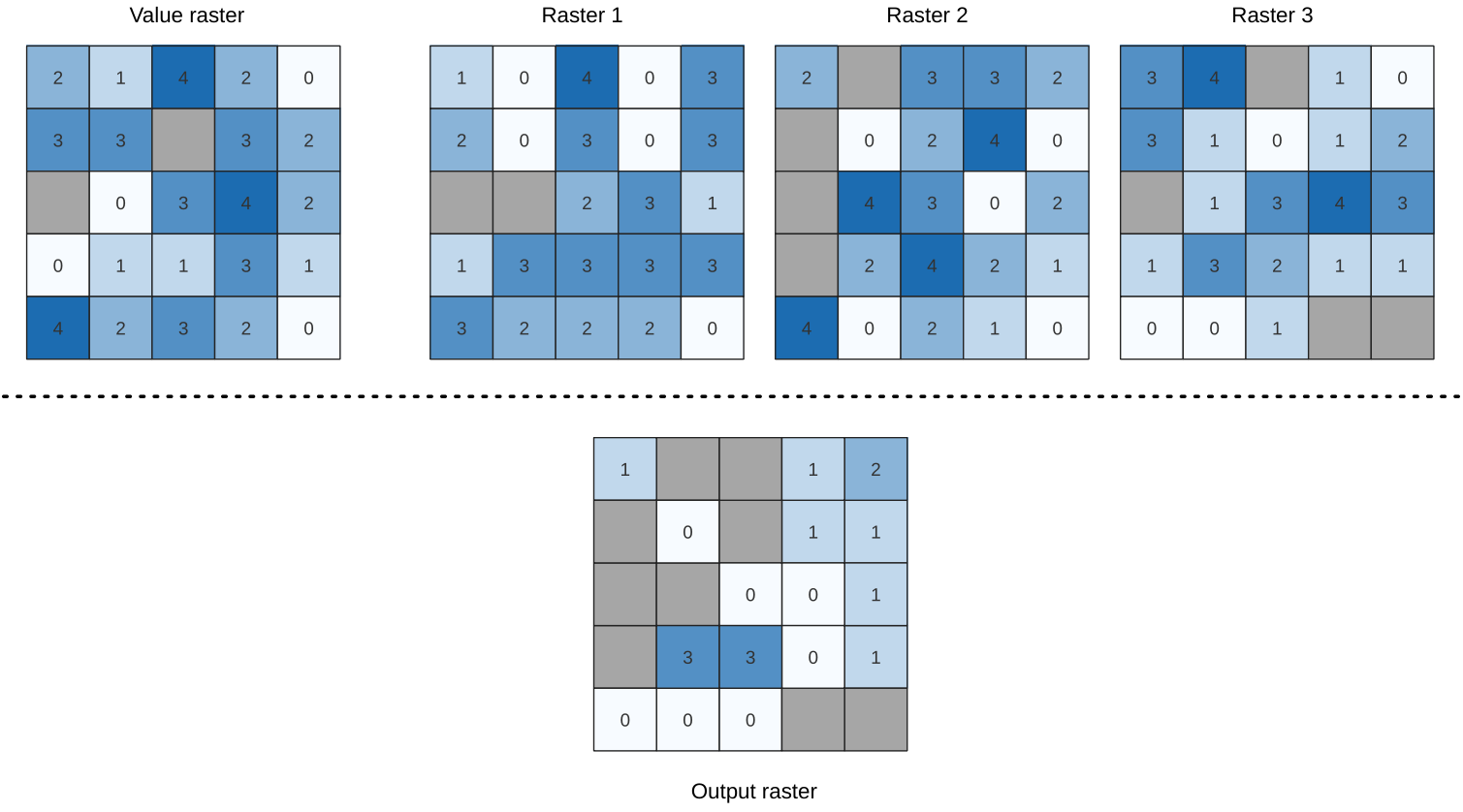Fig. 25.16 For each cell in the output raster, the value represents the number of times that the corresponding cells in the list of rasters are greater than the value raster. `NoData` cells (grey) are taken into account.

### 25.1.9.12.1. Parameters¶

#### 25.1.9.12.1.1. Basic parameters¶

Label

Name

Type

Description

Input value raster

`INPUT_VALUE_RASTER`

[raster]

The input value layer serves as reference layer for the sample layers

Value raster band

`INPUT_VALUE_RASTER_BAND`

[raster band]

Default: The first band of the raster layer

Select the band you want to use as sample

Input raster layers

`INPUT_RASTERS`

[raster] [list]

Raster layers to evaluate. If multiband rasters are used in the data raster stack, the algorithm will always perform the analysis on the first band of the rasters

Ignore NoData values

`IGNORE_NODATA`

[boolean]

Default: False

If unchecked, any NoData cells in the value raster or the data layer stack will result in a NoData cell in the output raster

Output layer

`OUTPUT`

[same as input]

Default: `[Save to temporary file]`

Specification of the output raster. One of:

• Save to a Temporary File

• Save to File…

Label

Name

Type

Description

Output no data value

Optional

`OUTPUT_NO_DATA_VALUE`

[number]

Default: -9999.0

Value to use for nodata in the output layer

### 25.1.9.12.2. Outputs¶

Label

Name

Type

Description

Output layer

`OUTPUT`

[raster]

Output raster layer containing the result

CRS authority identifier

`CRS_AUTHID`

[string]

The coordinate reference system of the output raster layer

Extent

`EXTENT`

[string]

The spatial extent of the output raster layer

Count of cells with equal value occurrences

`FOUND_LOCATIONS_COUNT`

[number]

Height in pixels

`HEIGHT_IN_PIXELS`

[number]

The number of rows in the output raster layer

Total pixel count

`TOTAL_PIXEL_COUNT`

[integer]

The count of pixels in the output raster layer

Mean frequency at valid cell locations

`MEAN_FREQUENCY_PER_LOCATION`

[number]

Count of value occurrences

`OCCURRENCE_COUNT`

[number]

Width in pixels

`WIDTH_IN_PIXELS`

[integer]

The number of columns in the output raster layer

### 25.1.9.12.3. Python code¶

Algorithm ID: `native:greaterthanfrequency`

```import processing
processing.run("algorithm_id", {parameter_dictionary})
```

The algorithm id is displayed when you hover over the algorithm in the Processing Toolbox. The parameter dictionary provides the parameter NAMEs and values. See Using processing algorithms from the console for details on how to run processing algorithms from the Python console.

## 25.1.9.13. Highest position in raster stack¶

Evaluates on a cell-by-cell basis the position of the raster with the highest value in a stack of rasters. Position counts start with 1 and range to the total number of input rasters. The order of the input rasters is relevant for the algorithm. If multiple rasters feature the highest value, the first raster will be used for the position value.

If multiband rasters are used in the data raster stack, the algorithm will always perform the analysis on the first band of the rasters - use GDAL to use other bands in the analysis. Any NoData cells in the raster layer stack will result in a NoData cell in the output raster unless the “ignore NoData” parameter is checked. The output NoData value can be set manually. The output rasters extent and resolution is defined by a reference raster layer and is always of `Int32` type.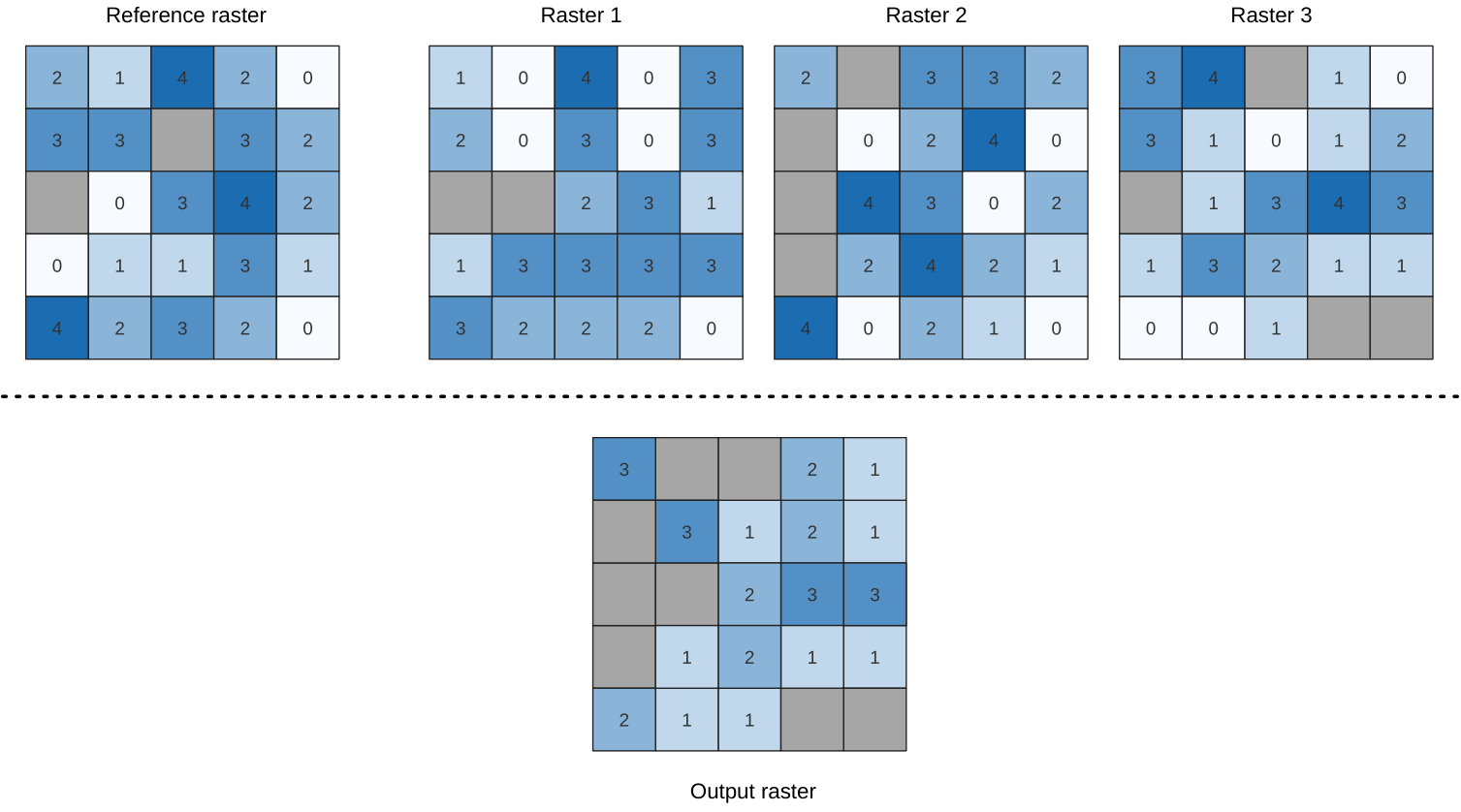### 25.1.9.13.1. Parameters¶

#### 25.1.9.13.1.1. Basic parameters¶

Label

Name

Type

Description

Input raster layers

`INPUT_RASTERS`

[raster] [list]

List of raster layers to compare with

Reference layer

`REFERENCE_LAYER`

[raster]

The reference layer for the output layer creation (extent, CRS, pixel dimensions)

Ignore NoData values

`IGNORE_NODATA`

[boolean]

Default: False

If unchecked, any NoData cells in the data layer stack will result in a NoData cell in the output raster

Output layer

`OUTPUT`

[raster]

Default: `[Save to temporary file]`

Specification of the output raster containing the result. One of:

• Save to a Temporary File

• Save to File…

Label

Name

Type

Description

Output no data value

`OUTPUT_NODATA_VALUE`

[number]

Default: -9999.0

Value to use for nodata in the output layer

### 25.1.9.13.2. Outputs¶

Label

Name

Type

Description

Output layer

`OUTPUT`

[raster]

Output raster layer containing the result

CRS authority identifier

`CRS_AUTHID`

[string]

The coordinate reference system of the output raster layer

Extent

`EXTENT`

[string]

The spatial extent of the output raster layer

Width in pixels

`WIDTH_IN_PIXELS`

[integer]

The number of columns in the output raster layer

Height in pixels

`HEIGHT_IN_PIXELS`

[integer]

The number of rows in the output raster layer

Total pixel count

`TOTAL_PIXEL_COUNT`

[integer]

The count of pixels in the output raster layer

### 25.1.9.13.3. Python code¶

Algorithm ID: `native:highestpositioninrasterstack`

```import processing
processing.run("algorithm_id", {parameter_dictionary})
```

The algorithm id is displayed when you hover over the algorithm in the Processing Toolbox. The parameter dictionary provides the parameter NAMEs and values. See Using processing algorithms from the console for details on how to run processing algorithms from the Python console.

## 25.1.9.14. Less than frequency¶

Evaluates on a cell-by-cell basis the frequency (number of times) the values of an input stack of rasters are less than the value of a value raster. The output raster extent and resolution is defined by the input raster layer and is always of `Int32` type.

If multiband rasters are used in the data raster stack, the algorithm will always perform the analysis on the first band of the rasters - use GDAL to use other bands in the analysis. The output NoData value can be set manually.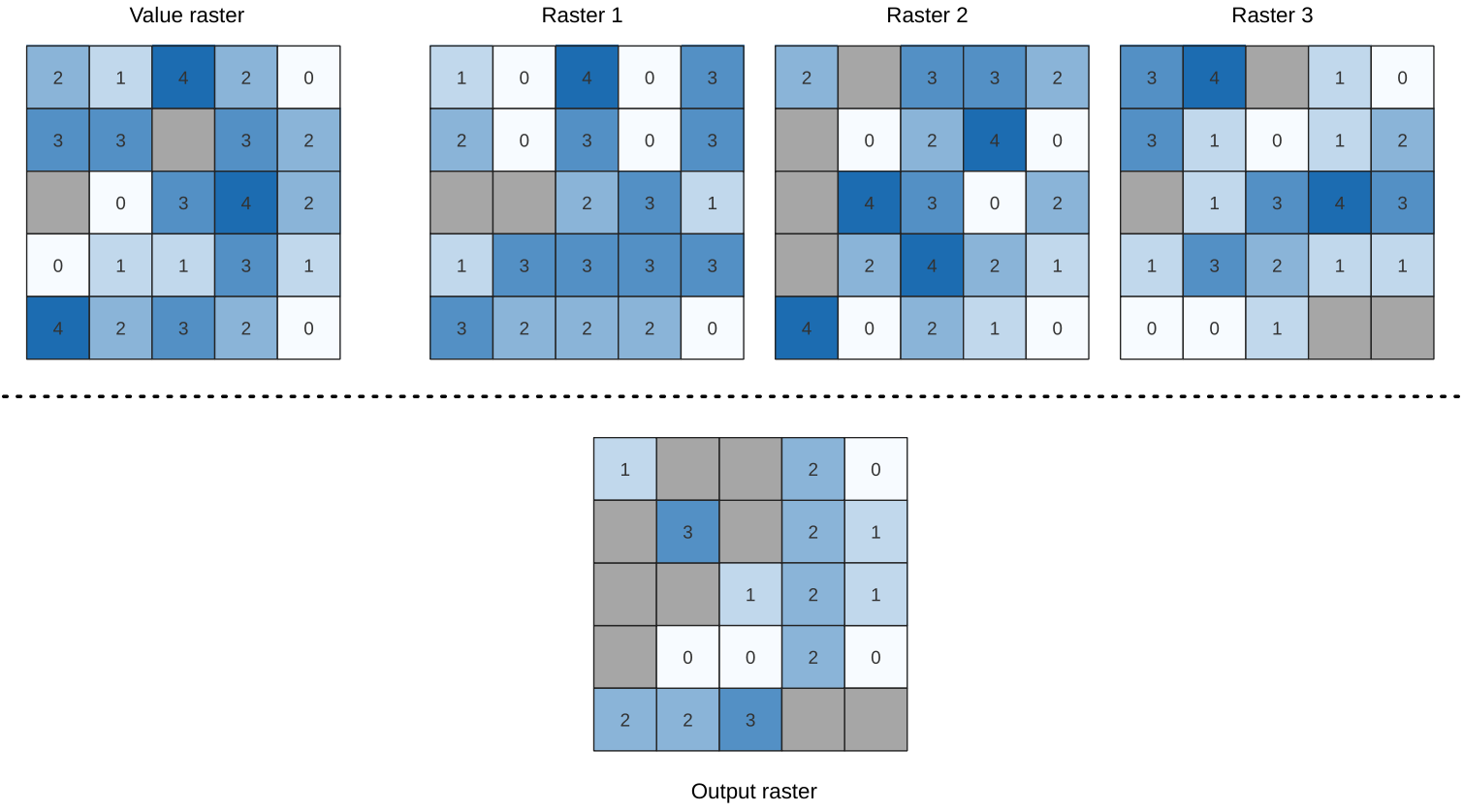Fig. 25.17 For each cell in the output raster, the value represents the number of times that the corresponding cells in the list of rasters are less than the value raster. `NoData` cells (grey) are taken into account.

### 25.1.9.14.1. Parameters¶

#### 25.1.9.14.1.1. Basic parameters¶

Label

Name

Type

Description

Input value raster

`INPUT_VALUE_RASTER`

[raster]

The input value layer serves as reference layer for the sample layers

Value raster band

`INPUT_VALUE_RASTER_BAND`

[raster band]

Default: The first band of the raster layer

Select the band you want to use as sample

Input raster layers

`INPUT_RASTERS`

[raster] [list]

Raster layers to evaluate. If multiband rasters are used in the data raster stack, the algorithm will always perform the analysis on the first band of the rasters

Ignore NoData values

`IGNORE_NODATA`

[boolean]

Default: False

If unchecked, any NoData cells in the value raster or the data layer stack will result in a NoData cell in the output raster

Output layer

`OUTPUT`

[same as input]

Default: `[Save to temporary file]`

Specification of the output raster. One of:

• Save to a Temporary File

• Save to File…

Label

Name

Type

Description

Output no data value

Optional

`OUTPUT_NO_DATA_VALUE`

[number]

Default: -9999.0

Value to use for nodata in the output layer

### 25.1.9.14.2. Outputs¶

Label

Name

Type

Description

Output layer

`OUTPUT`

[raster]

Output raster layer containing the result

CRS authority identifier

`CRS_AUTHID`

[string]

The coordinate reference system of the output raster layer

Extent

`EXTENT`

[string]

The spatial extent of the output raster layer

Count of cells with equal value occurrences

`FOUND_LOCATIONS_COUNT`

[number]

Height in pixels

`HEIGHT_IN_PIXELS`

[number]

The number of rows in the output raster layer

Total pixel count

`TOTAL_PIXEL_COUNT`

[integer]

The count of pixels in the output raster layer

Mean frequency at valid cell locations

`MEAN_FREQUENCY_PER_LOCATION`

[number]

Count of value occurrences

`OCCURRENCE_COUNT`

[number]

Width in pixels

`WIDTH_IN_PIXELS`

[integer]

The number of columns in the output raster layer

### 25.1.9.14.3. Python code¶

Algorithm ID: `native:lessthanfrequency`

```import processing
processing.run("algorithm_id", {parameter_dictionary})
```

The algorithm id is displayed when you hover over the algorithm in the Processing Toolbox. The parameter dictionary provides the parameter NAMEs and values. See Using processing algorithms from the console for details on how to run processing algorithms from the Python console.

## 25.1.9.15. Lowest position in raster stack¶

Evaluates on a cell-by-cell basis the position of the raster with the lowest value in a stack of rasters. Position counts start with 1 and range to the total number of input rasters. The order of the input rasters is relevant for the algorithm. If multiple rasters feature the highest value, the first raster will be used for the position value.

If multiband rasters are used in the data raster stack, the algorithm will always perform the analysis on the first band of the rasters - use GDAL to use other bands in the analysis. Any NoData cells in the raster layer stack will result in a NoData cell in the output raster unless the “ignore NoData” parameter is checked. The output NoData value can be set manually. The output rasters extent and resolution is defined by a reference raster layer and is always of `Int32` type.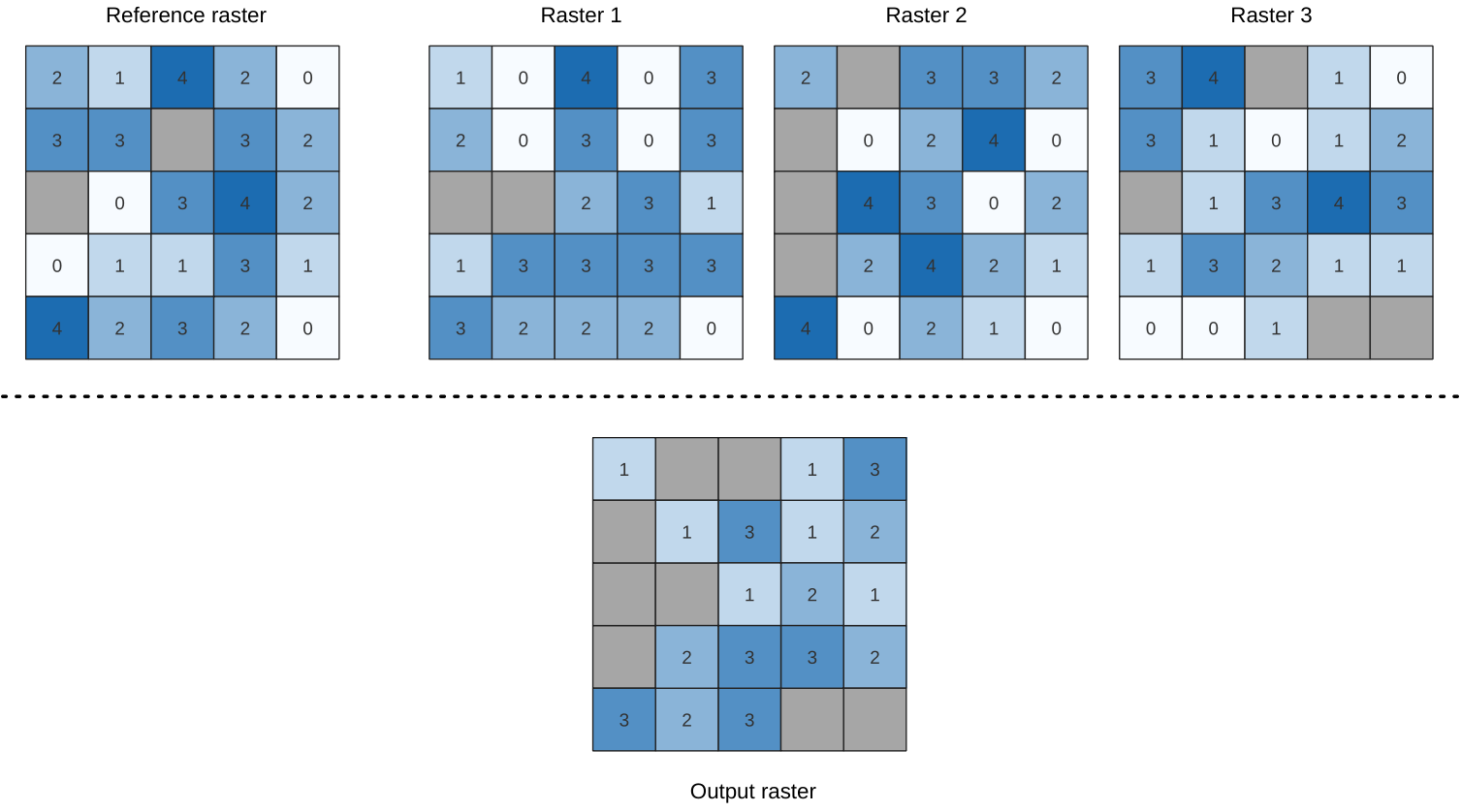### 25.1.9.15.1. Parameters¶

#### 25.1.9.15.1.1. Basic parameters¶

Label

Name

Type

Description

Input raster layers

`INPUT_RASTERS`

[raster] [list]

List of raster layers to compare with

Reference layer

`REFERENCE_LAYER`

[raster]

The reference layer for the output layer creation (extent, CRS, pixel dimensions)

Ignore NoData values

`IGNORE_NODATA`

[boolean]

Default: False

If unchecked, any NoData cells in the data layer stack will result in a NoData cell in the output raster

Output layer

`OUTPUT`

[raster]

Default: `[Save to temporary file]`

Specification of the output raster containing the result. One of:

• Save to a Temporary File

• Save to File…

Label

Name

Type

Description

Output no data value

`OUTPUT_NODATA_VALUE`

[number]

Default: -9999.0

Value to use for nodata in the output layer

### 25.1.9.15.2. Outputs¶

Label

Name

Type

Description

Output layer

`OUTPUT`

[raster]

Output raster layer containing the result

CRS authority identifier

`CRS_AUTHID`

[string]

The coordinate reference system of the output raster layer

Extent

`EXTENT`

[string]

The spatial extent of the output raster layer

Width in pixels

`WIDTH_IN_PIXELS`

[integer]

The number of columns in the output raster layer

Height in pixels

`HEIGHT_IN_PIXELS`

[integer]

The number of rows in the output raster layer

Total pixel count

`TOTAL_PIXEL_COUNT`

[integer]

The count of pixels in the output raster layer

### 25.1.9.15.3. Python code¶

Algorithm ID: `native:lowestpositioninrasterstack`

```import processing
processing.run("algorithm_id", {parameter_dictionary})
```

The algorithm id is displayed when you hover over the algorithm in the Processing Toolbox. The parameter dictionary provides the parameter NAMEs and values. See Using processing algorithms from the console for details on how to run processing algorithms from the Python console.

## 25.1.9.16. Raster boolean AND¶

Calculates the boolean `AND` for a set of input rasters. If all of the input rasters have a non-zero value for a pixel, that pixel will be set to `1` in the output raster. If any of the input rasters have `0` values for the pixel it will be set to `0` in the output raster.

The reference layer parameter specifies an existing raster layer to use as a reference when creating the output raster. The output raster will have the same extent, CRS, and pixel dimensions as this layer.

By default, a nodata pixel in ANY of the input layers will result in a nodata pixel in the output raster. If the Treat nodata values as false option is checked, then nodata inputs will be treated the same as a `0` input value.

### 25.1.9.16.1. Parameters¶

#### 25.1.9.16.1.1. Basic parameters¶

Label

Name

Type

Description

Input layers

`INPUT`

[raster] [list]

List of input raster layers

Reference layer

`REF_LAYER`

[raster]

The reference layer to create the output layer from (extent, CRS, pixel dimensions)

Treat nodata values as false

`NODATA_AS_FALSE`

[boolean]

Default: False

Treat nodata values in the input files as 0 when performing the operation

Output layer

`OUTPUT`

[raster]

Default: `[Save to temporary file]`

Specification of the output raster containing the result. One of:

• Save to a Temporary File

• Save to File…

Label

Name

Type

Description

Output no data value

`NO_DATA`

[number]

Default: -9999.0

Value to use for nodata in the output layer

Output data type

`DATA_TYPE`

[enumeration]

Default: 5

Output raster data type. Options:

• 0 — Byte

• 1 — Int16

• 2 — UInt16

• 3 — UInt32

• 4 — Int32

• 5 — Float32

• 6 — Float64

• 7 — CInt16

• 8 — CInt32

• 9 — CFloat32

• 10 — CFloat64

### 25.1.9.16.2. Outputs¶

Label

Name

Type

Description

Extent

`EXTENT`

[extent]

The extent of the output raster layer

CRS authority identifier

`CRS_AUTHID`

[crs]

The coordinate reference system of the output raster layer

Width in pixels

`WIDTH_IN_PIXELS`

[integer]

The number of columns in the output raster layer

Height in pixels

`HEIGHT_IN_PIXELS`

[integer]

The number of rows in the output raster layer

Total pixel count

`TOTAL_PIXEL_COUNT`

[integer]

The count of pixels in the output raster layer

NODATA pixel count

`NODATA_PIXEL_COUNT`

[integer]

The count of nodata pixels in the output raster layer

True pixel count

`TRUE_PIXEL_COUNT`

[integer]

The count of True pixels (value = 1) in the output raster layer

False pixel count

`FALSE_PIXEL_COUNT`

[integer]

The count of False pixels (value = 0) in the output raster layer

Output layer

`OUTPUT`

[raster]

Output raster layer containing the result

### 25.1.9.16.3. Python code¶

Algorithm ID: `native:rasterbooleanand`

```import processing
processing.run("algorithm_id", {parameter_dictionary})
```

The algorithm id is displayed when you hover over the algorithm in the Processing Toolbox. The parameter dictionary provides the parameter NAMEs and values. See Using processing algorithms from the console for details on how to run processing algorithms from the Python console.

## 25.1.9.17. Raster boolean OR¶

Calculates the boolean `OR` for a set of input rasters. If all of the input rasters have a zero value for a pixel, that pixel will be set to `0` in the output raster. If any of the input rasters have `1` values for the pixel it will be set to `1` in the output raster.

The reference layer parameter specifies an existing raster layer to use as a reference when creating the output raster. The output raster will have the same extent, CRS, and pixel dimensions as this layer.

By default, a nodata pixel in ANY of the input layers will result in a nodata pixel in the output raster. If the Treat nodata values as false option is checked, then nodata inputs will be treated the same as a `0` input value.

### 25.1.9.17.1. Parameters¶

#### 25.1.9.17.1.1. Basic parameters¶

Label

Name

Type

Description

Input layers

`INPUT`

[raster] [list]

List of input raster layers

Reference layer

`REF_LAYER`

[raster]

The reference layer to create the output layer from (extent, CRS, pixel dimensions)

Treat nodata values as false

`NODATA_AS_FALSE`

[boolean]

Default: False

Treat nodata values in the input files as 0 when performing the operation

Output layer

`OUTPUT`

[raster]

Default: `[Save to temporary file]`

Specification of the output raster containing the result. One of:

• Save to a Temporary File

• Save to File…

Label

Name

Type

Description

Output no data value

`NO_DATA`

[number]

Default: -9999.0

Value to use for nodata in the output layer

Output data type

`DATA_TYPE`

[enumeration]

Default: 5

Output raster data type. Options:

• 0 — Byte

• 1 — Int16

• 2 — UInt16

• 3 — UInt32

• 4 — Int32

• 5 — Float32

• 6 — Float64

• 7 — CInt16

• 8 — CInt32

• 9 — CFloat32

• 10 — CFloat64

### 25.1.9.17.2. Outputs¶

Label

Name

Type

Description

Extent

`EXTENT`

[extent]

The extent of the output raster layer

CRS authority identifier

`CRS_AUTHID`

[crs]

The coordinate reference system of the output raster layer

Width in pixels

`WIDTH_IN_PIXELS`

[integer]

The number of columns in the output raster layer

Height in pixels

`HEIGHT_IN_PIXELS`

[integer]

The number of rows in the output raster layer

Total pixel count

`TOTAL_PIXEL_COUNT`

[integer]

The count of pixels in the output raster layer

NODATA pixel count

`NODATA_PIXEL_COUNT`

[integer]

The count of nodata pixels in the output raster layer

True pixel count

`TRUE_PIXEL_COUNT`

[integer]

The count of True pixels (value = 1) in the output raster layer

False pixel count

`FALSE_PIXEL_COUNT`

[integer]

The count of False pixels (value = 0) in the output raster layer

Output layer

`OUTPUT`

[raster]

Output raster layer containing the result

### 25.1.9.17.3. Python code¶

Algorithm ID: `native:rasterbooleanor`

```import processing
processing.run("algorithm_id", {parameter_dictionary})
```

The algorithm id is displayed when you hover over the algorithm in the Processing Toolbox. The parameter dictionary provides the parameter NAMEs and values. See Using processing algorithms from the console for details on how to run processing algorithms from the Python console.

## 25.1.9.18. Raster calculator¶

Performs algebraic operations using raster layers.

The resulting layer will have its values computed according to an expression. The expression can contain numerical values, operators and references to any of the layers in the current project.

Note

When using the calculator in The batch processing interface or from the QGIS Python console the files to use have to be specified. The corresponding layers are referred using the base name of the file (without the full path). For instance, if using a layer at `path/to/my/rasterfile.tif`, the first band of that layer will be referred as `rasterfile.tif@1`.

### 25.1.9.18.1. Parameters¶

Label

Name

Type

Description

Layers

GUI only

Shows the list of all raster layers loaded in the legend. These can be used to fill the expression box (double click to add). Raster layers are referred by their name and the number of the band: `layer_name@band_number`. For instance, the first band from a layer named `DEM` will be referred as `DEM@1`.

Operators

GUI only

Contains some calculator like buttons that can be used to fill the expression box.

Expression

`EXPRESSION`

[string]

Expression that will be used to calculate the output raster layer. You can use the operator buttons provided to type directly the expression in this box.

Predefined expressions

GUI only

You can use the predefined `NDVI` expression or you can define new expressions for calculations. The Add… button loads a defined expression (and lets you set the parameters). The Save… button lets you define a new expression.

Reference layer(s) (used for automated extent, cellsize, and CRS)

Optional

`LAYERS`

[raster] [list]

Layer(s) that will be used to fetch extent, cell size and CRS. By choosing the layer in this box you avoid filling in all the other parameters by hand. Raster layers are referred by their name and the number of the band: `layer_name@band_number`. For instance, the first band from a layer named `DEM` will be referred as `DEM@1`.

Cell size (use 0 or empty to set it automatically)

Optional

`CELLSIZE`

[number]

Cell size of the output raster layer. If the cell size is not specified, the minimum cell size of the selected reference layer(s) will be used. The cell size will be the same for the X and Y axes.

Output extent

Optional

`EXTENT`

[extent]

Extent (xmin, xmax, ymin, ymax) of the output raster layer. If the extent is not specified, the minimum extent that covers all the selected reference layers will be used.

Output CRS

Optional

`CRS`

[crs]

CRS of the output raster layer. If the output CRS is not specified, the CRS of the first reference layer will be used.

Output

`OUTPUT`

[raster]

Default: `[Save to temporary file]`

Specification of the output raster. One of:

• Save to a Temporary File

• Save to File…

### 25.1.9.18.2. Outputs¶

Label

Name

Type

Description

Output

`OUTPUT`

[raster]

Output raster file with the calculated values.

### 25.1.9.18.3. Python code¶

Algorithm ID: `qgis:rastercalculator`

```import processing
processing.run("algorithm_id", {parameter_dictionary})
```

The algorithm id is displayed when you hover over the algorithm in the Processing Toolbox. The parameter dictionary provides the parameter NAMEs and values. See Using processing algorithms from the console for details on how to run processing algorithms from the Python console.

## 25.1.9.19. Raster layer properties¶

`NEW in 3.20`

Returns basic properties of the given raster layer, including the extent, size in pixels and dimensions of pixels (in map units), number of bands, and no data value.

This algorithm is intended for use as a means of extracting these useful properties to use as the input values to other algorithms in a model - e.g. to allow to pass an existing raster’s pixel sizes over to a GDAL raster algorithm.

### 25.1.9.19.1. Parameters¶

Label

Name

Type

Description

Input layer

`INPUT`

[raster]

Input raster layer

Band number

Optional

`BAND`

[raster band]

Default: Not set

Whether to also return properties of a specific band. If a band is specified, the noData value for the selected band is also returned.

### 25.1.9.19.2. Outputs¶

Label

Name

Type

Description

Number of bands in raster

`BAND_COUNT`

[number]

The number of bands in the raster

CRS authority identifier

`CRS_AUTHID`

[string]

Extent

`EXTENT`

[string]

The raster layer extent in the CRS

Band has a NoData value set

`HAS_NODATA_VALUE`

[Boolean]

Indicates whether the raster layer has a value set for NODATA pixels in the selected band

Height in pixels

`HEIGHT_IN_PIXELS`

[number]

The number of columns in the raster layer

Band NoData value

`NODATA_VALUE`

[number]

The value (if set) of the NoData pixels in the selected band

Pixel size (height) in map units

`PIXEL_HEIGHT`

[number]

Vertical size in map units of the pixel

Pixel size (width) in map units

`PIXEL_WIDTH`

[number]

Horizontal size in map units of the pixel

Width in pixels

`WIDTH_IN_PIXELS`

[number]

The number of rows in the raster layer

Maximum x-coordinate

`X_MAX`

[number]

Minimum x-coordinate

`X_MIN`

[number]

Maximum y-coordinate

`Y_MAX`

[number]

Minimum y-coordinate

`Y_MIN`

[number]

### 25.1.9.19.3. Python code¶

Algorithm ID: `native:rasterlayerproperties`

```import processing
processing.run("algorithm_id", {parameter_dictionary})
```

The algorithm id is displayed when you hover over the algorithm in the Processing Toolbox. The parameter dictionary provides the parameter NAMEs and values. See Using processing algorithms from the console for details on how to run processing algorithms from the Python console.

## 25.1.9.20. Raster layer statistics¶

Calculates basic statistics from the values in a given band of the raster layer. The output is loaded in the Processing ► Results viewer menu.

### 25.1.9.20.1. Parameters¶

Label

Name

Type

Description

Input layer

`INPUT`

[raster]

Input raster layer

Band number

`BAND`

[raster band]

Default: The first band of the input layer

If the raster is multiband, choose the band you want to get statistics for.

Statistics

`OUTPUT_HTML_FILE`

[html]

Default: `[Save to temporary file]`

Specification of the output file:

• Skip Output

• Save to a Temporary File

• Save to File…

### 25.1.9.20.2. Outputs¶

Label

Name

Type

Description

Maximum value

`MAX`

[number]

Mean value

`MEAN`

[number]

Minimum value

`MIN`

[number]

Statistics

`OUTPUT_HTML_FILE`

[html]

The output file contains the following information:

• Analyzed file: path of the raster layer

• Minimum value: minimum value of the raster

• Maximum value: maximum value of the raster

• Range: difference between the maximum and minimum values

• Sum: total sum of the values

• Mean value: mean of the values

• Standard deviation: standard deviation of the values

• Sum of the squares: sum of the squared differences of each observation from the overall mean

Range

`RANGE`

[number]

Standard deviation

`STD_DEV`

[number]

Sum

`SUM`

[number]

Sum of the squares

`SUM_OF_SQUARES`

[number]

### 25.1.9.20.3. Python code¶

Algorithm ID: `qgis:rasterlayerstatistics`

```import processing
processing.run("algorithm_id", {parameter_dictionary})
```

The algorithm id is displayed when you hover over the algorithm in the Processing Toolbox. The parameter dictionary provides the parameter NAMEs and values. See Using processing algorithms from the console for details on how to run processing algorithms from the Python console.

## 25.1.9.21. Raster layer unique values report¶

Returns the count and area of each unique value in a given raster layer.

### 25.1.9.21.1. Parameters¶

Label

Name

Type

Description

Input layer

`INPUT`

[raster]

Input raster layer

Band number

`BAND`

[raster band]

Default: The first band of the input layer

If the raster is multiband, choose the band you want to get statistics for.

Unique values report

`OUTPUT_HTML_FILE`

[file]

Default: `[Save to temporary file]`

Specification of the output file:

• Skip Output

• Save to a Temporary File

• Save to File…

Unique values table

`OUTPUT_TABLE`

[table]

Default: `[Skip output]`

Specification of the table for unique values:

• Skip Output

• Create Temporary Layer (`TEMPORARY_OUTPUT`)

• Save to File…

• Save to Geopackage…

• Save to Database Table…

The file encoding can also be changed here.

### 25.1.9.21.2. Outputs¶

Label

Name

Type

Description

CRS authority identifier

`CRS_AUTHID`

[crs]

Extent

`EXTENT`

[extent]

Height in pixels

`HEIGHT_IN_PIXELS`

[number]

NODATA pixel count

`NODATA_PIXEL_COUNT`

[number]

Total pixel count

`TOTAL_PIXEL_COUNT`

[number]

Unique values report

`OUTPUT_HTML_FILE`

[html]

The output HTML file contains the following information:

• Analyzed file: the path of the raster layer

• Extent: xmin, ymin, xmax, ymax coordinates of the extent

• Projection: projection of the layer

• Width in pixels: number of columns and pixel width size

• Height in pixels: number of rows and pixel width size

• Total pixel count: count of all the pixels

• NODATA pixel count: count of pixels with NODATA value

Unique values table

`OUTPUT_TABLE`

[table]

A table with three columns:

• value: pixel value

• count: count of pixels with this value

• m2: total area in square meters of pixels with this value.

Width in pixels

`WIDTH_IN_PIXELS`

[number]

### 25.1.9.21.3. Python code¶

Algorithm ID: `native:rasterlayeruniquevaluesreport`

```import processing
processing.run("algorithm_id", {parameter_dictionary})
```

The algorithm id is displayed when you hover over the algorithm in the Processing Toolbox. The parameter dictionary provides the parameter NAMEs and values. See Using processing algorithms from the console for details on how to run processing algorithms from the Python console.

## 25.1.9.22. Raster layer zonal statistics¶

Calculates statistics for a raster layer’s values, categorized by zones defined in another raster layer.

Zonal statistics

### 25.1.9.22.1. Parameters¶

#### 25.1.9.22.1.1. Basic parameters¶

Label

Name

Type

Description

Input Layer

`INPUT`

[raster]

Input raster layer

Band number

`BAND`

[raster band]

Default: The first band of the raster layer

If the raster is multiband choose the band for which you want to calculate the statistics.

Zones layer

`ZONES`

[raster]

Raster layer defining zones. Zones are given by contiguous pixels having the same pixel value.

Zones band number

`ZONES_BAND`

[raster band]

Default: The first band of the raster layer

If the raster is multiband, choose the band that defines the zones

Statistics

`OUTPUT_TABLE`

[table]

Default: `[Create temporary layer]`

Specification of the output report. One of:

• Create Temporary Layer (`TEMPORARY_OUTPUT`)

• Save to File…

• Save to Geopackage…

• Save to Database Table…

The file encoding can also be changed here.

Label

Name

Type

Description

Reference layer

Optional

`REF_LAYER`

[enumeration]

Default: 0

Raster layer used to calculate the centroids that will be used as reference when determining the zones in the output layer. One of:

• 0 — Input layer: zones are determined by sampling the zone raster layer value at the centroid of each pixel from the source raster layer

• 1 — Zones layer: the input raster layer will be sampled at the centroid of each pixel from the zones raster layer

### 25.1.9.22.2. Outputs¶

Label

Name

Type

Description

CRS authority identifier

`CRS_AUTHID`

[crs]

Extent

`EXTENT`

[extent]

Height in pixels

`HEIGHT_IN_PIXELS`

[number]

NODATA pixel count

`NODATA_PIXEL_COUNT`

[number]

Statistics

`OUTPUT_TABLE`

[table]

The output layer contains the following information for each zone:

• Area: the area in square raster units in the zone;

• Sum: the total sum of the pixel values in the zone;

• Count: the number of pixels in the zone;

• Min: the minimum pixel value in the zone;

• Max: the maximum pixel value in the zone;

• Mean: the mean of the pixel values in the zone;

Total pixel count

`TOTAL_PIXEL_COUNT`

[number]

Width in pixels

`WIDTH_IN_PIXELS`

[number]

### 25.1.9.22.3. Python code¶

Algorithm ID: `native:rasterlayerzonalstats`

```import processing
processing.run("algorithm_id", {parameter_dictionary})
```

The algorithm id is displayed when you hover over the algorithm in the Processing Toolbox. The parameter dictionary provides the parameter NAMEs and values. See Using processing algorithms from the console for details on how to run processing algorithms from the Python console.

## 25.1.9.23. Raster surface volume¶

Calculates the volume under a raster surface relative to a given base level. This is mainly useful for Digital Elevation Models (DEM).

### 25.1.9.23.1. Parameters¶

Label

Name

Type

Description

INPUT layer

`INPUT`

[raster]

Input raster, representing a surface

Band number

`BAND`

[raster band]

Default: The first band of the raster layer

If the raster is multiband, choose the band that shall define the surface.

Base level

`LEVEL`

[number]

Default: 0.0

Define a base or reference value. This base is used in the volume calculation according to the `Method` parameter (see below).

Method

`METHOD`

[enumeration]

Default: 0

Define the method for the volume calculation given by the difference between the raster pixel value and the `Base level`. Options:

• 0 — Count Only Above Base Level: only pixels above the base level will add to the volume.

• 1 — Count Only Below Base Level: only pixels below the base level will add to the volume.

• 2 — Subtract Volumes Below Base level: pixels above the base level will add to the volume, pixels below the base level will subtract from the volume.

• 3 — Add Volumes Below Base level: Add the volume regardless whether the pixel is above or below the base level. This is equivalent to sum the absolute values of the difference between the pixel value and the base level.

Surface volume report

`OUTPUT_HTML_FILE`

[html]

Default: `[Save to temporary file]`

Specification of the output HTML report. One of:

• Skip Output

• Create Temporary Layer (`TEMPORARY_OUTPUT`)

• Save to File…

• Save to Geopackage…

• Save to Database Table…

The file encoding can also be changed here.

Surface volume table

`OUTPUT_TABLE`

[table]

Default: `[Skip output]`

Specification of the output table. One of:

• Skip Output

• Create Temporary Layer (`TEMPORARY_OUTPUT`)

• Save to File…

• Save to Geopackage…

• Save to Database Table…

The file encoding can also be changed here.

### 25.1.9.23.2. Outputs¶

Label

Name

Type

Description

Volume

`VOLUME`

[number]

The calculated volume

Area

`AREA`

[number]

The area in square map units

Pixel_count

`PIXEL_COUNT`

[number]

The total number of pixels that have been analyzed

Surface volume report

`OUTPUT_HTML_FILE`

[html]

The output report (containing volume, area and pixel count) in HTML format

Surface volume table

`OUTPUT_TABLE`

[table]

The output table (containing volume, area and pixel count)

### 25.1.9.23.3. Python code¶

Algorithm ID: `native:rastersurfacevolume`

```import processing
processing.run("algorithm_id", {parameter_dictionary})
```

The algorithm id is displayed when you hover over the algorithm in the Processing Toolbox. The parameter dictionary provides the parameter NAMEs and values. See Using processing algorithms from the console for details on how to run processing algorithms from the Python console.

## 25.1.9.24. Reclassify by layer¶

Reclassifies a raster band by assigning new class values based on the ranges specified in a vector table.

### 25.1.9.24.1. Parameters¶

#### 25.1.9.24.1.1. Basic parameters¶

Label

Name

Type

Description

Raster layer

`INPUT_RASTER`

[raster]

Raster layer to reclassify

Band number

`RASTER_BAND`

[raster band]

Default: The first band of the raster layer

If the raster is multiband, choose the band you want to reclassify.

Layer containing class breaks

`INPUT_TABLE`

[vector: any]

Vector layer containing the values to use for classification.

Minimum class value field

`MIN_FIELD`

[tablefield: numeric]

Field with the minimum value of the range for the class.

Maximum class value field

`MAX_FIELD`

[tablefield: numeric]

Field with the maximum value of the range for the class.

Output value field

`VALUE_FIELD`

[tablefield: numeric]

Field with the value that will be assigned to the pixels that fall in the class (between the corresponding min and max values).

Reclassified raster

`OUTPUT`

[raster]

Default: `[Save to temporary file]`

Specification of the output raster. One of:

• Save to a Temporary File

• Save to File…

Label

Name

Type

Description

Output no data value

`NO_DATA`

[number]

Default: -9999.0

Value to apply to no data values.

Range boundaries

`RANGE_BOUNDARIES`

[enumeration]

Default: 0

Defines comparison rules for the classification. Options:

• 0 — min < value <= max

• 1 — min <= value < max

• 2 — min <= value <= max

• 3 — min < value < max

Use no data when no range matches value

`NODATA_FOR_MISSING`

[boolean]

Default: False

Applies the no data value to band values that do not fall in any class. If False, the original value is kept.

Output data type

`DATA_TYPE`

[enumeration]

Default: 5

Defines the format of the output raster file. Options:

• 0 — Byte

• 1 — Int16

• 2 — UInt16

• 3 — UInt32

• 4 — Int32

• 5 — Float32

• 6 — Float64

• 7 — CInt16

• 8 — CInt32

• 9 — CFloat32

• 10 — CFloat64

### 25.1.9.24.2. Outputs¶

Label

Name

Type

Description

Reclassified raster

`OUTPUT`

[raster]

Output raster layer with reclassified band values

### 25.1.9.24.3. Python code¶

Algorithm ID: `native:reclassifybylayer`

```import processing
processing.run("algorithm_id", {parameter_dictionary})
```

The algorithm id is displayed when you hover over the algorithm in the Processing Toolbox. The parameter dictionary provides the parameter NAMEs and values. See Using processing algorithms from the console for details on how to run processing algorithms from the Python console.

## 25.1.9.25. Reclassify by table¶

Reclassifies a raster band by assigning new class values based on the ranges specified in a fixed table.

### 25.1.9.25.1. Parameters¶

#### 25.1.9.25.1.1. Basic parameters¶

Label

Name

Type

Description

Raster layer

`INPUT_RASTER`

[raster]

Raster layer to reclassify

Band number

`RASTER_BAND`

[raster band]

Default: 1

Raster band for which you want to recalculate values.

Reclassification table

`TABLE`

[table]

A 3-columns table to fill with the values to set the boundaries of each class (`Minimum` and `Maximum`) and the new `Value` to assign to the band values that fall in the class.

Reclassified raster

`OUTPUT`

[raster]

Default: `[Save to temporary file]`

Specification of the output raster layer. One of:

• Save to a Temporary File

• Save to File…

Label

Name

Type

Description

Output no data value

`NO_DATA`

[number]

Default: -9999.0

Value to apply to no data values.

Range boundaries

`RANGE_BOUNDARIES`

[enumeration]

Default: 0

Defines comparison rules for the classification. Options:

• 0 — min < value <= max

• 1 — min <= value < max

• 2 — min <= value <= max

• 3 — min < value < max

Use no data when no range matches value

`NODATA_FOR_MISSING`

[boolean]

Default: False

Applies the no data value to band values that do not fall in any class. If False, the original value is kept.

Output data type

`DATA_TYPE`

[enumeration]

Default: 5

Defines the format of the output raster file. Options:

• 0 — Byte

• 1 — Int16

• 2 — UInt16

• 3 — UInt32

• 4 — Int32

• 5 — Float32

• 6 — Float64

• 7 — CInt16

• 8 — CInt32

• 9 — CFloat32

• 10 — CFloat64

### 25.1.9.25.2. Outputs¶

Label

Name

Type

Description

Reclassified raster

`OUTPUT`

[raster]

Output raster layer with reclassified band values

### 25.1.9.25.3. Python code¶

Algorithm ID: `native:reclassifybytable`

```import processing
processing.run("algorithm_id", {parameter_dictionary})
```

The algorithm id is displayed when you hover over the algorithm in the Processing Toolbox. The parameter dictionary provides the parameter NAMEs and values. See Using processing algorithms from the console for details on how to run processing algorithms from the Python console.

## 25.1.9.26. Rescale raster¶

Rescales raster layer to a new value range, while preserving the shape (distribution) of the raster’s histogram (pixel values). Input values are mapped using a linear interpolation from the source raster’s minimum and maximum pixel values to the destination minimum and miximum pixel range.

By default the algorithm preserves the original NODATA value, but there is an option to override it.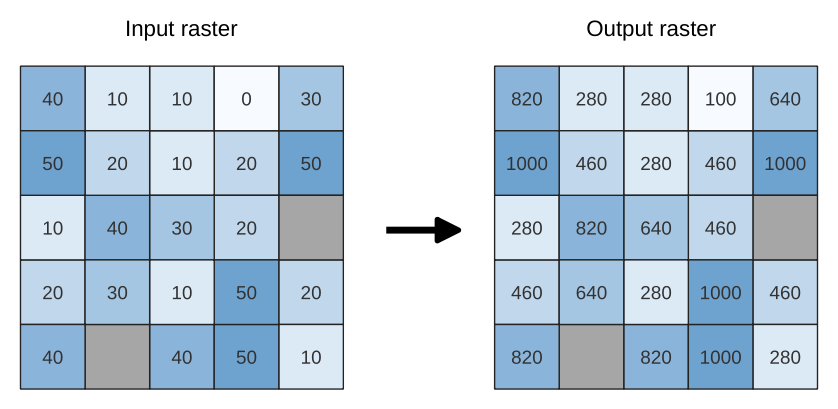Fig. 25.18 Rescaling values of a raster layer from [0 - 50] to [100 - 1000]

### 25.1.9.26.1. Parameters¶

Label

Name

Type

Description

Input Raster

`INPUT`

[raster]

Raster layer to use for rescaling

Band number

`Band`

[raster band]

Default: The first band of the input layer

If the raster is multiband, choose a band.

New minimum value

`MINIMUM`

[number]

Default value: 0.0

Minimum pixel value to use in the rescaled layer

New maximum value

`MAXIMUM`

[number]

Default value: 255.0

Maximum pixel value to use in the rescaled layer

New NODATA value

Optional

`NODATA`

[number]

Default value: Not set

Value to assign to the NODATA pixels. If unset, original NODATA values are preserved.

Rescaled

`OUTPUT`

[raster]

Default: `[Save to temporary file]`

Specification of the output raster layer. One of:

• Save to a Temporary File

• Save to File…

### 25.1.9.26.2. Outputs¶

Label

Name

Type

Description

Rescaled

`OUTPUT`

[raster]

Output raster layer with rescaled band values

### 25.1.9.26.3. Python code¶

Algorithm ID: `native:rescaleraster`

```import processing
processing.run("algorithm_id", {parameter_dictionary})
```

The algorithm id is displayed when you hover over the algorithm in the Processing Toolbox. The parameter dictionary provides the parameter NAMEs and values. See Using processing algorithms from the console for details on how to run processing algorithms from the Python console.

## 25.1.9.27. Round raster¶

Rounds the cell values of a raster dataset according to the specified number of decimals.

Alternatively, a negative number of decimal places may be used to round values to powers of a base n. For example, with a Base value n of 10 and Decimal places of -1, the algorithm rounds cell values to multiples of 10, -2 rounds to multiples of 100, and so on. Arbitrary base values may be chosen, the algorithm applies the same multiplicative principle. Rounding cell values to multiples of a base n may be used to generalize raster layers.

The algorithm preserves the data type of the input raster. Therefore byte/integer rasters can only be rounded to multiples of a base n, otherwise a warning is raised and the raster gets copied as byte/integer raster.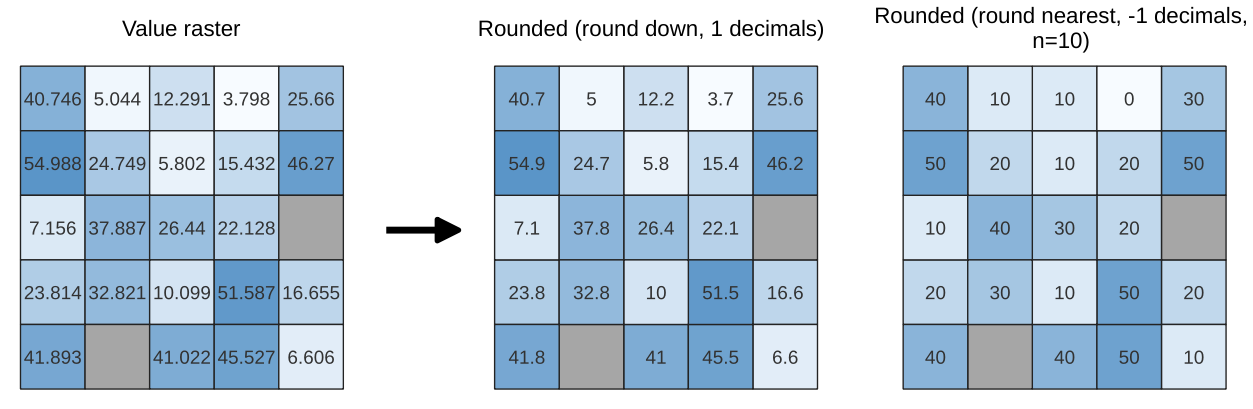Fig. 25.19 Rounding values of a raster

### 25.1.9.27.1. Parameters¶

#### 25.1.9.27.1.1. Basic parameters¶

Label

Name

Type

Description

Input raster

`INPUT`

[raster]

The raster to process.

Band number

`BAND`

[number]

Default: 1

The band of the raster

Rounding direction

`ROUNDING_DIRECTION`

[list]

Default: 1

How to choose the target rounded value. Options are:

• 0 — Round up

• 1 — Round to nearest

• 2 — Round down

Number of decimals places

`DECIMAL_PLACES`

[number]

Default: 2

Number of decimals places to round to. Use negative values to round cell values to a multiple of a base n

Output raster

`OUTPUT`

[raster]

Default: `[Save to temporary file]`

Specification of the output file. One of:

• Save to a Temporary File

• Save to File…

Label

Name

Type

Description

Base n for rounding to multiples of n

`BASE_N`

[number]

Default: 10

When the `DECIMAL_PLACES` parameter is negative, raster values are rounded to multiples of the base n value

### 25.1.9.27.2. Outputs¶

Label

Name

Type

Description

Output raster

`OUTPUT`

[raster]

The output raster layer with values rounded for the selected band.

### 25.1.9.27.3. Python code¶

Algorithm ID: `native:roundrastervalues`

```import processing
processing.run("algorithm_id", {parameter_dictionary})
```

The algorithm id is displayed when you hover over the algorithm in the Processing Toolbox. The parameter dictionary provides the parameter NAMEs and values. See Using processing algorithms from the console for details on how to run processing algorithms from the Python console.

## 25.1.9.28. Sample raster values¶

Extracts raster values at the point locations. If the raster layer is multiband, each band is sampled.

The attribute table of the resulting layer will have as many new columns as the raster layer band count.

### 25.1.9.28.1. Parameters¶

Label

Name

Type

Description

Input Layer

`INPUT`

[vector: point]

Point vector layer to use for sampling

Raster Layer

`RASTERCOPY`

[raster]

Raster layer to sample at the given point locations.

Output column prefix

`COLUMN_PREFIX`

[string]

Default: ‘SAMPLE_’

Prefix for the names of the added columns.

Sampled

Optional

`OUTPUT`

[vector: point]

Default: `[Create temporary layer]`

Specify the output layer containing the sampled values. One of:

• Create Temporary Layer (`TEMPORARY_OUTPUT`)

• Save to File…

• Save to Geopackage…

• Save to Database Table…

The file encoding can also be changed here.

### 25.1.9.28.2. Outputs¶

Label

Name

Type

Description

Sampled

`OUTPUT`

[vector: point]

The output layer containing the sampled values.

### 25.1.9.28.3. Python code¶

Algorithm ID: `native:rastersampling`

```import processing
processing.run("algorithm_id", {parameter_dictionary})
```

The algorithm id is displayed when you hover over the algorithm in the Processing Toolbox. The parameter dictionary provides the parameter NAMEs and values. See Using processing algorithms from the console for details on how to run processing algorithms from the Python console.

## 25.1.9.29. Zonal histogram¶

Appends fields representing counts of each unique value from a raster layer contained within polygon features.

The output layer attribute table will have as many fields as the unique values of the raster layer that intersects the polygon(s).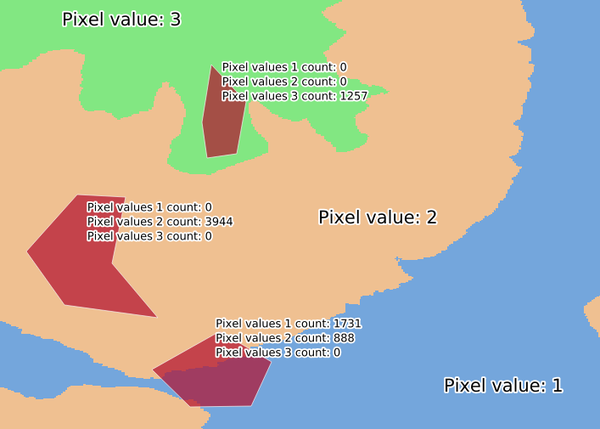Fig. 25.20 Raster layer histogram example

### 25.1.9.29.1. Parameters¶

Label

Name

Type

Description

Raster layer

`INPUT_RASTER`

[raster]

Input raster layer.

Band number

`RASTER_BAND`

[raster band]

Default: The first band of the input layer

If the raster is multiband, choose a band.

Vector layer containing zones

`INPUT_VECTOR`

[vector: polygon]

Vector polygon layer that defines the zones.

Output column prefix

`COLUMN_PREFIX`

Optional

[string]

Default: ‘HISTO_’

Prefix for the output columns names.

Output zones

`OUTPUT`

[vector: polygon]

Default: `[Create temporary layer]`

Specify the output vector polygon layer. One of:

• Create Temporary Layer (`TEMPORARY_OUTPUT`)

• Save to File…

• Save to Geopackage…

• Save to Database Table…

The file encoding can also be changed here.

### 25.1.9.29.2. Outputs¶

Label

Name

Type

Description

Output zones

`OUTPUT`

[vector: polygon]

The output vector polygon layer.

### 25.1.9.29.3. Python code¶

Algorithm ID: `native:zonalhistogram`

```import processing
processing.run("algorithm_id", {parameter_dictionary})
```

The algorithm id is displayed when you hover over the algorithm in the Processing Toolbox. The parameter dictionary provides the parameter NAMEs and values. See Using processing algorithms from the console for details on how to run processing algorithms from the Python console.

## 25.1.9.30. Zonal statistics¶

Calculates statistics of a raster layer for each feature of an overlapping polygon vector layer.

### 25.1.9.30.1. Parameters¶

Label

Name

Type

Description

Input layer

`INPUT`

[vector: polygon]

Vector polygon layer that contains the zones.

Raster layer

`INPUT_RASTER`

[raster]

Input raster layer.

Raster band

`RASTER_BAND`

[raster band]

Default: The first band of the input layer

If the raster is multiband, choose a band for the statistics.

Output column prefix

`COLUMN_PREFIX`

[string]

Default: ‘_’

Prefix for the output columns names.

Statistics to calculate

`STATISTICS`

[enumeration] [list]

Default: [0,1,2]

List of statistical operator for the output. Options:

• 0 — Count

• 1 — Sum

• 2 — Mean

• 3 — Median

• 4 — St. dev.

• 5 — Minimum

• 6 — Maximum

• 7 — Range

• 8 — Minority

• 9 — Majority

• 10 — Variety

• 11 — Variance

Zonal Statistics

`OUTPUT`

[vector: polygon]

Default: `[Create temporary layer]`

Specify the output vector polygon layer. One of:

• Create Temporary Layer (`TEMPORARY_OUTPUT`)

• Save to File…

• Save to Geopackage…

• Save to Database Table…

• Append to Layer…

The file encoding can also be changed here.

### 25.1.9.30.2. Outputs¶

Label

Name

Type

Description

Zonal Statistics

`OUTPUT`

[vector: polygon]

The zone vector layer with added statistics.

### 25.1.9.30.3. Python code¶

Algorithm ID: `native:zonalstatisticsfb`

```import processing
processing.run("algorithm_id", {parameter_dictionary})
```

The algorithm id is displayed when you hover over the algorithm in the Processing Toolbox. The parameter dictionary provides the parameter NAMEs and values. See Using processing algorithms from the console for details on how to run processing algorithms from the Python console.